Anzeige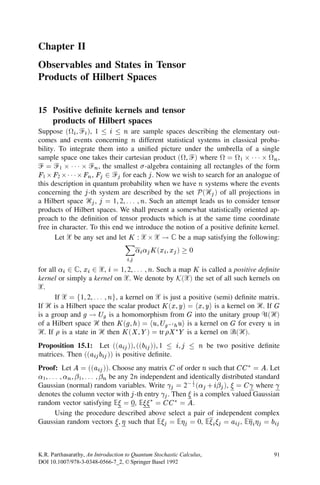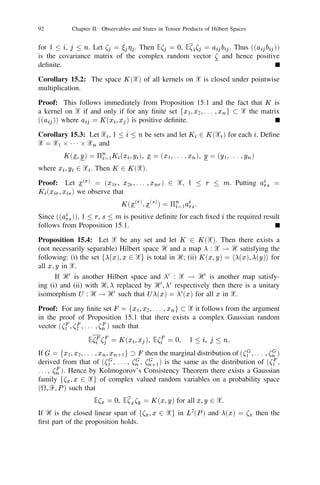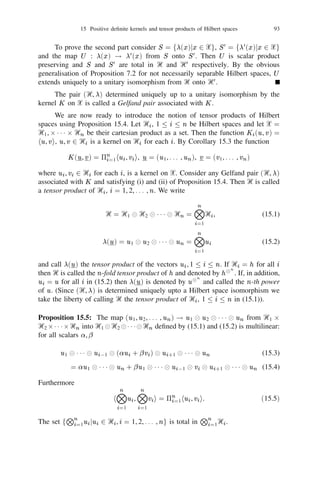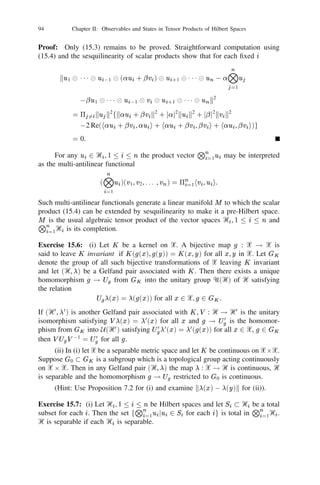Anzeige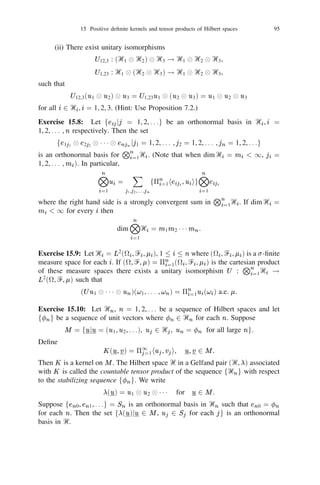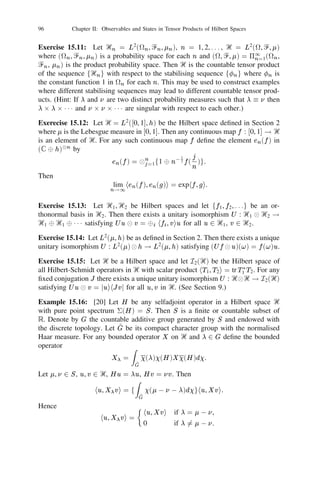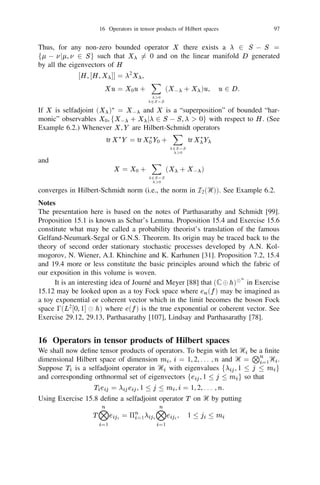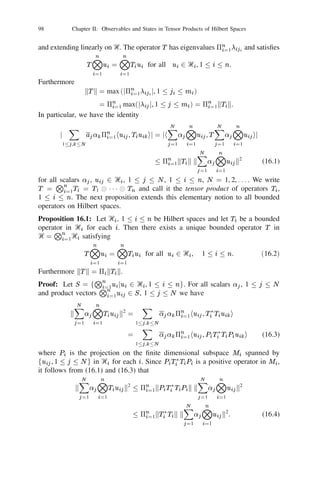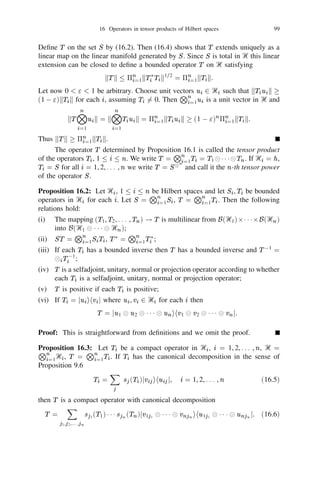Anzeige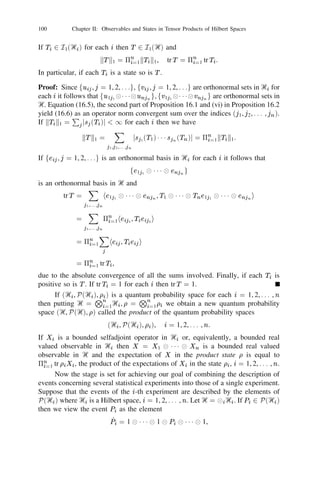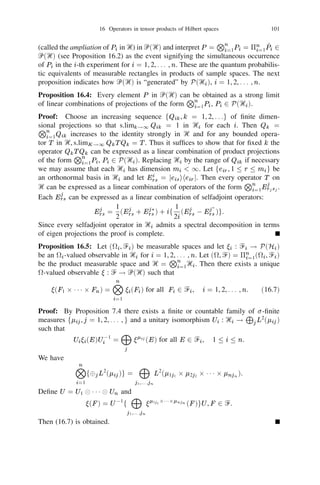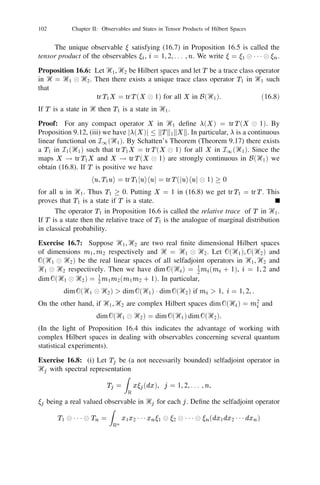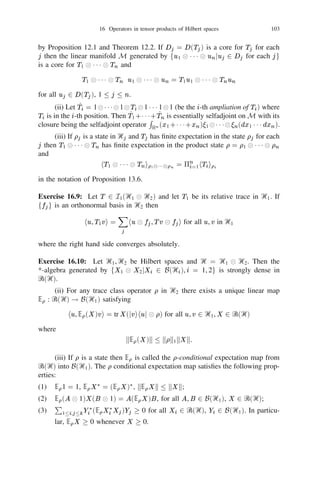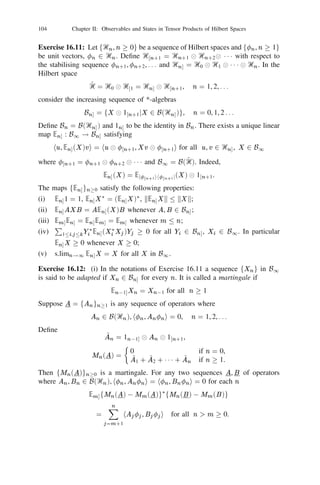Anzeige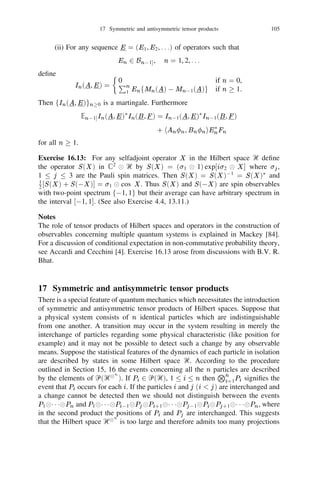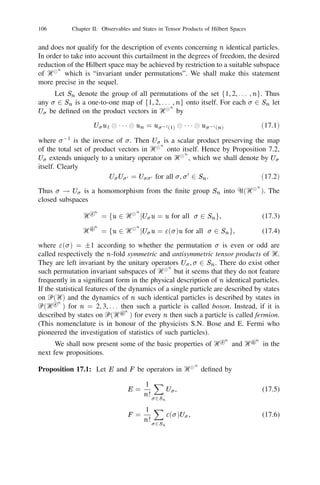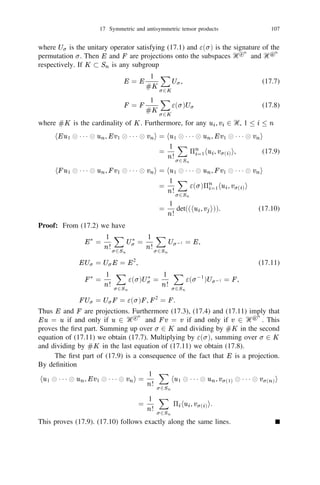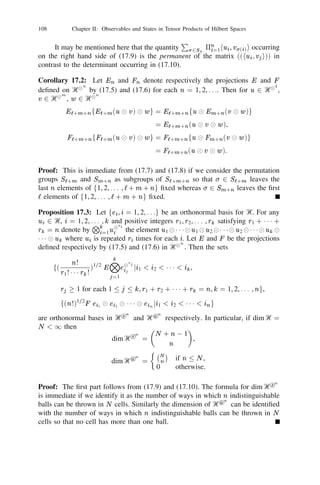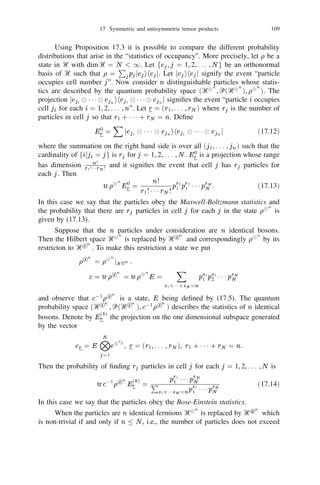Anzeige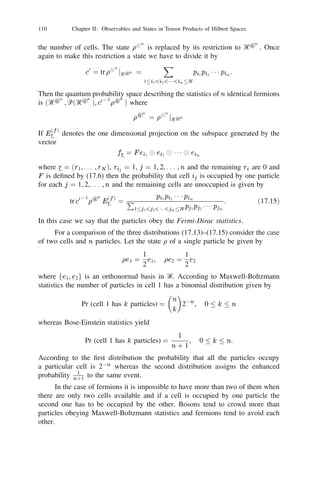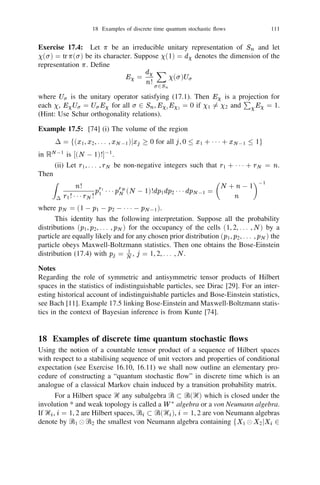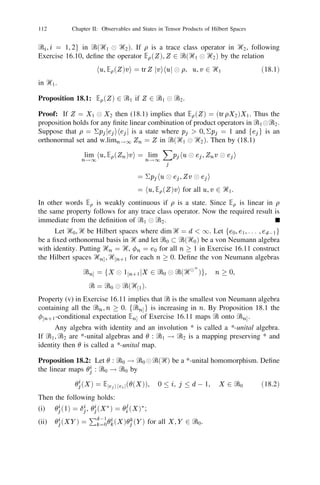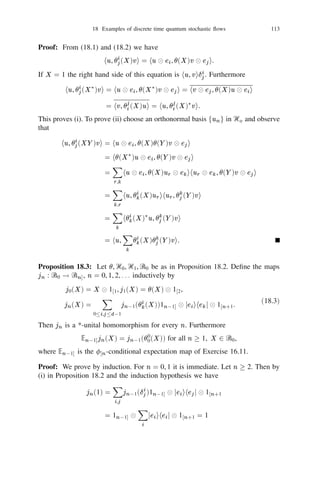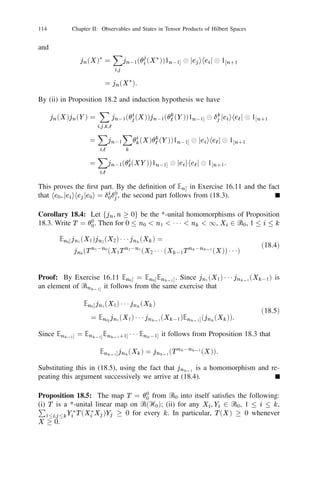Anzeige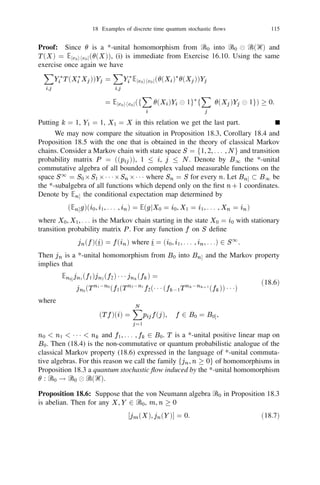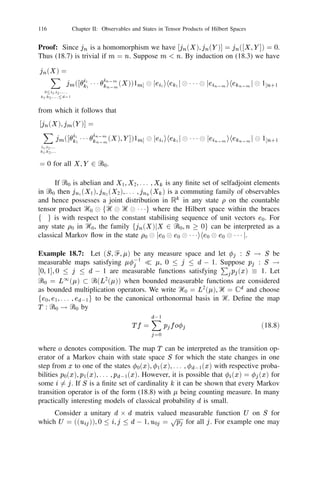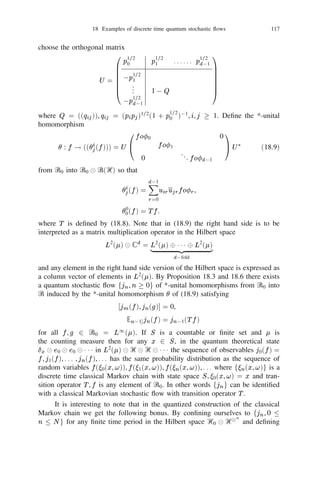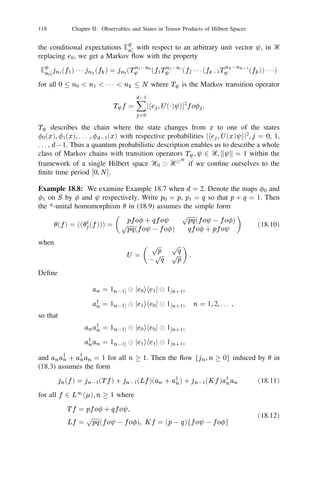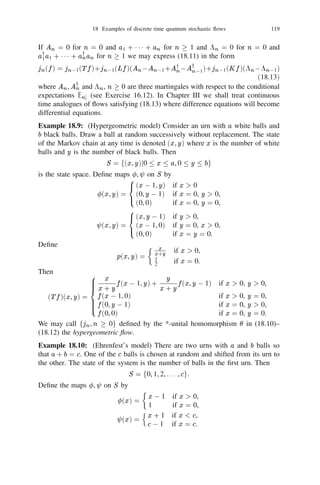Anzeige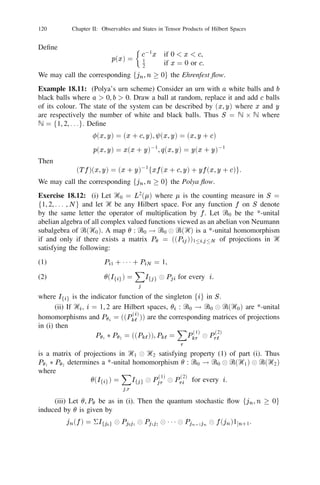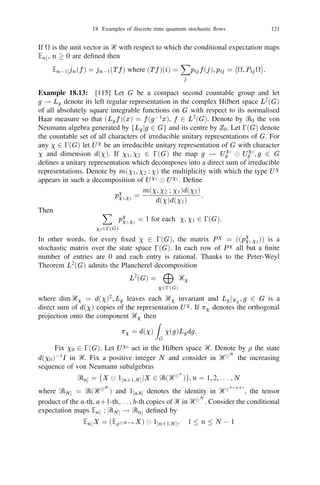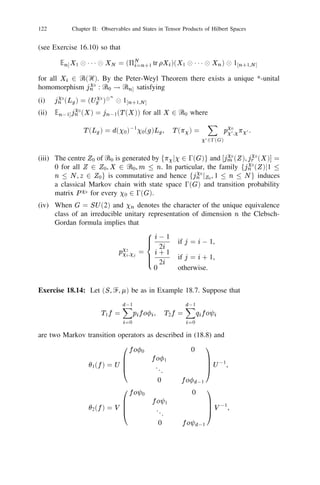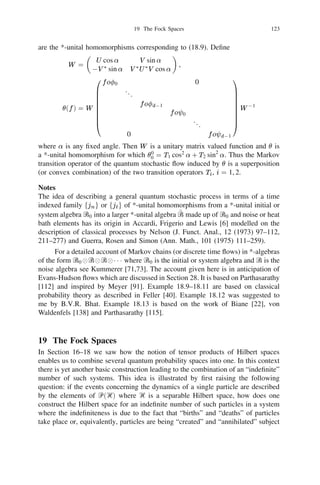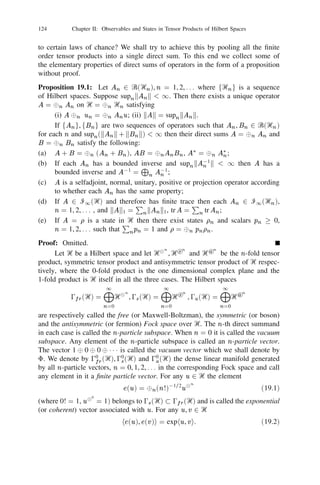Anzeige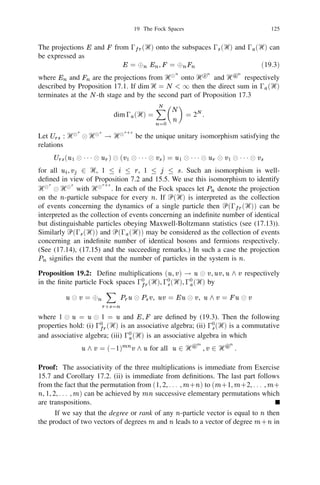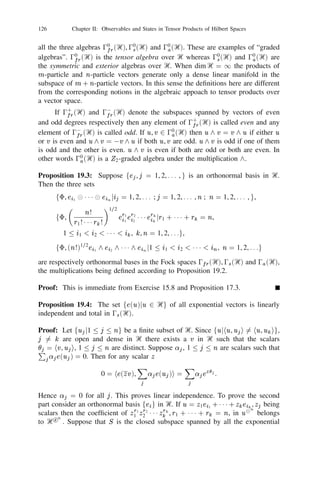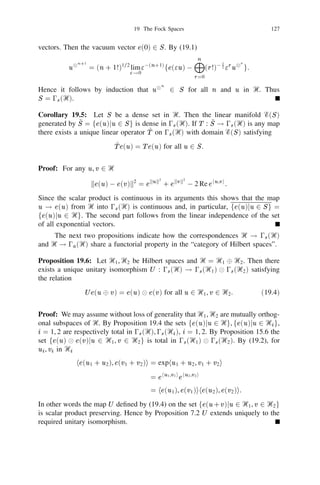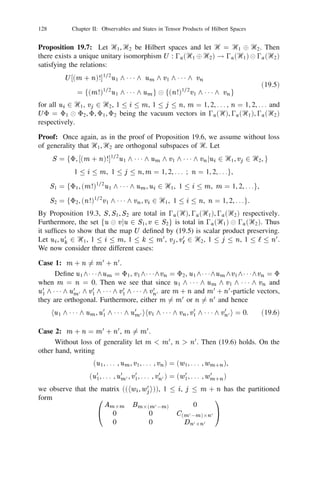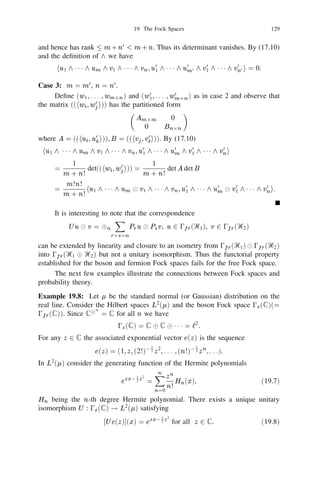Anzeige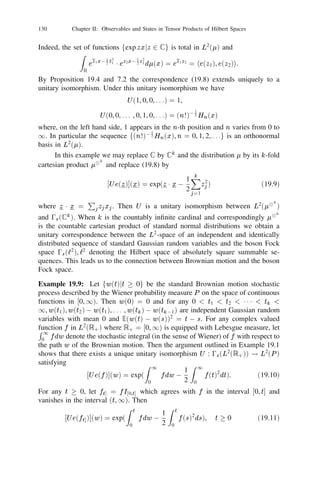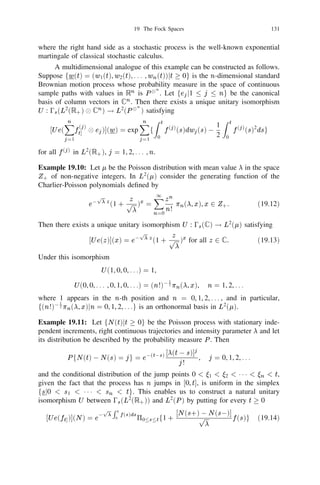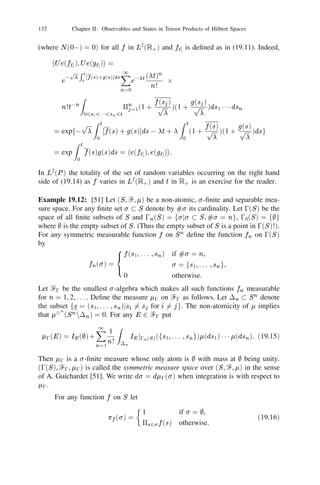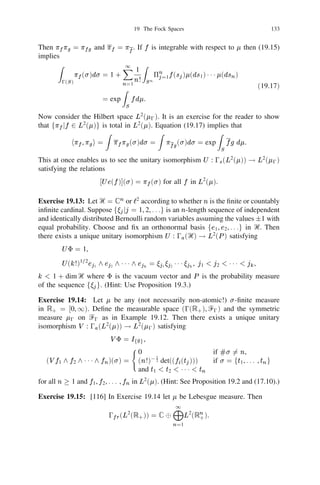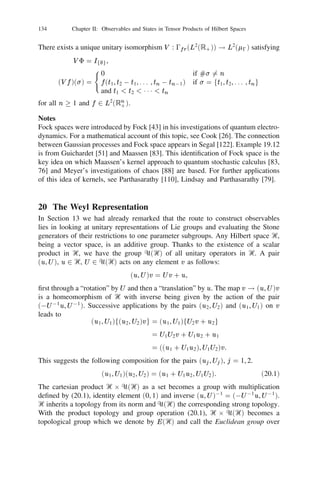Anzeige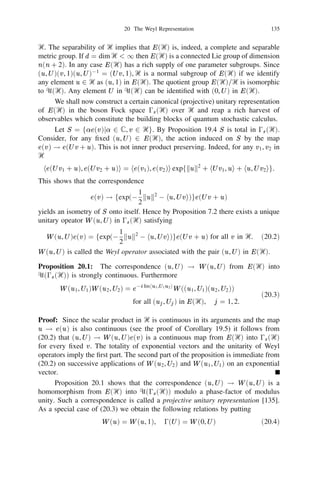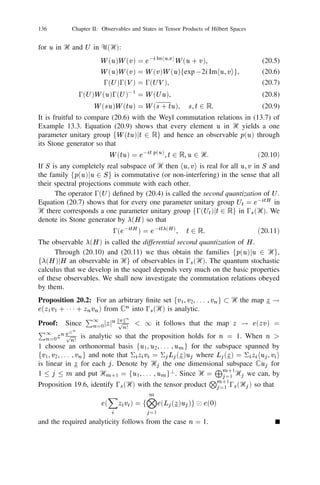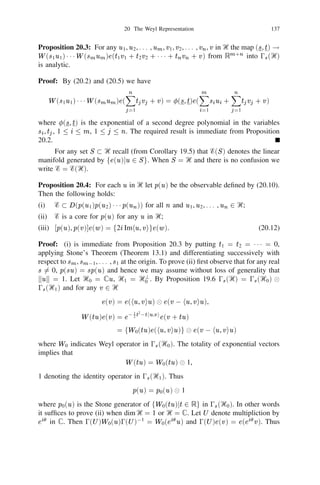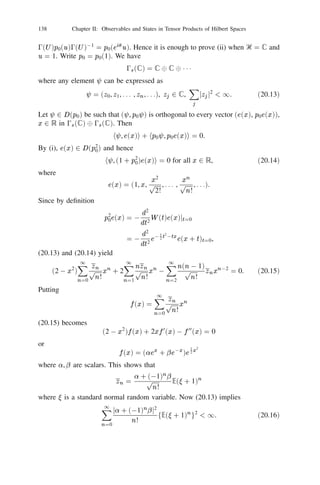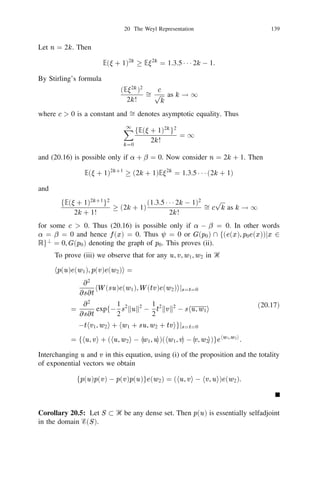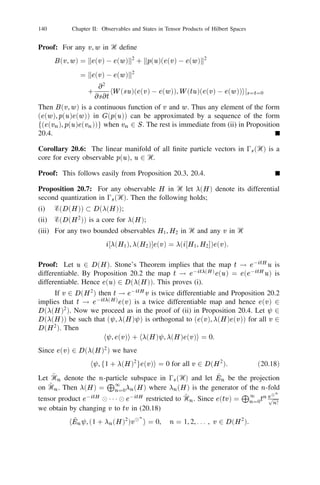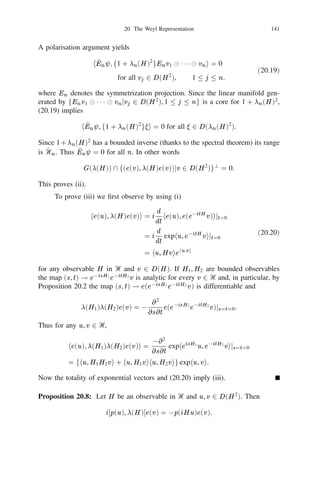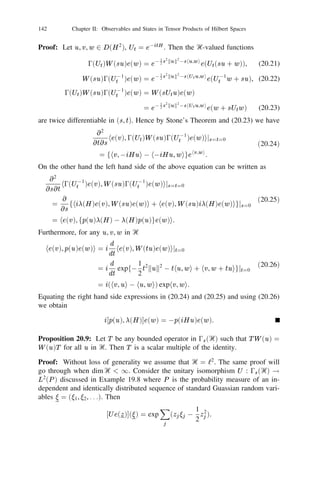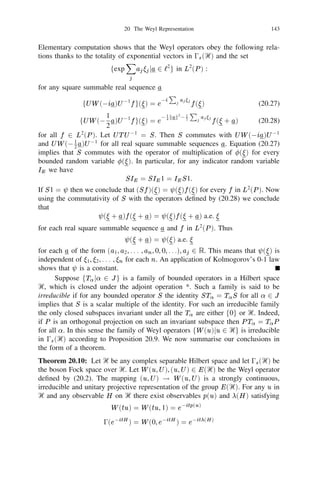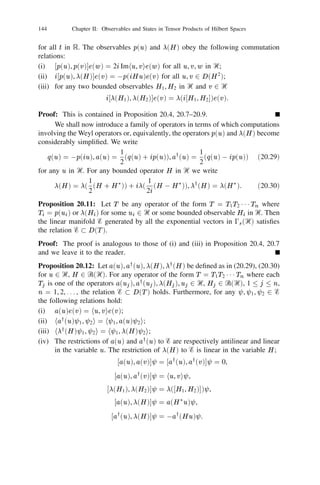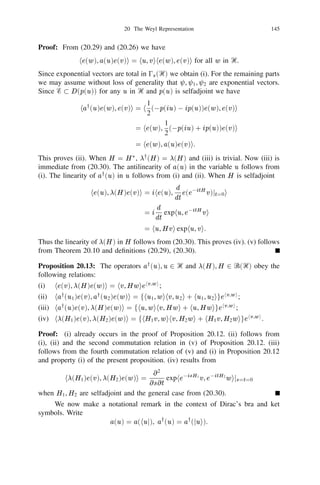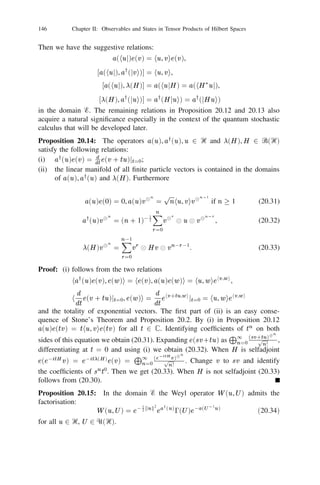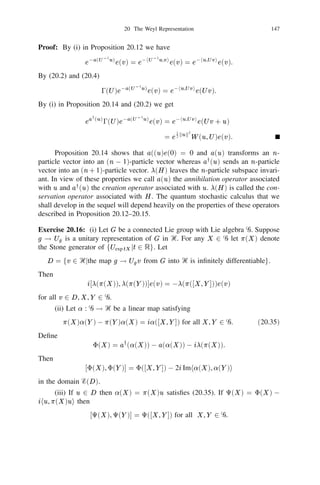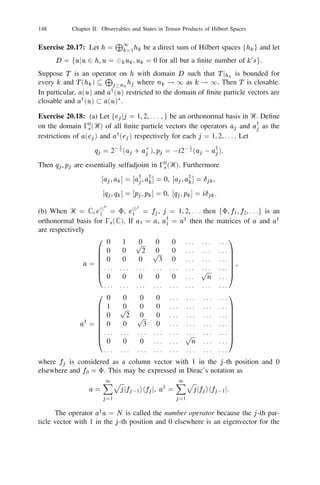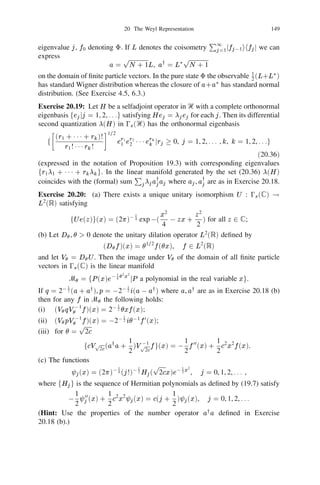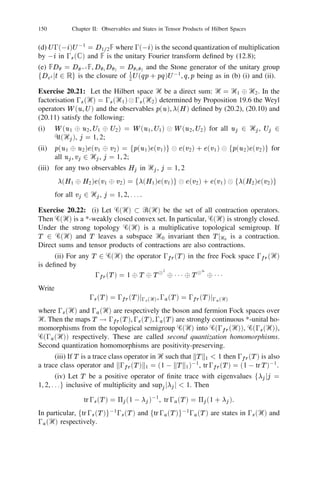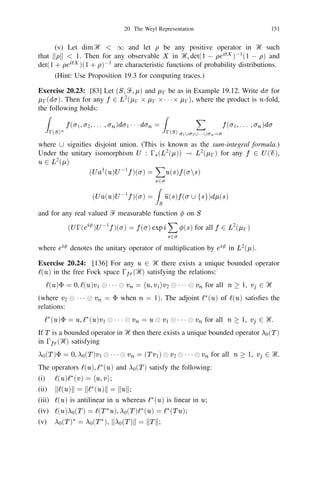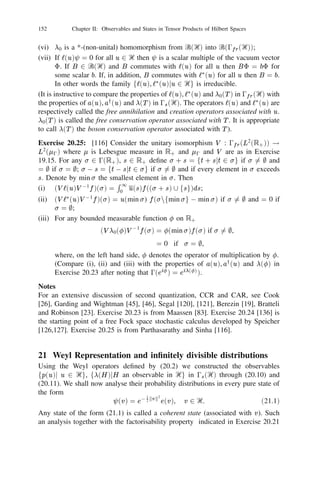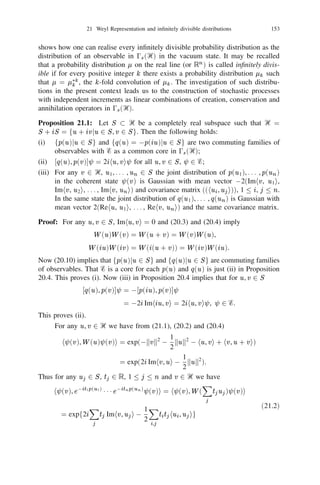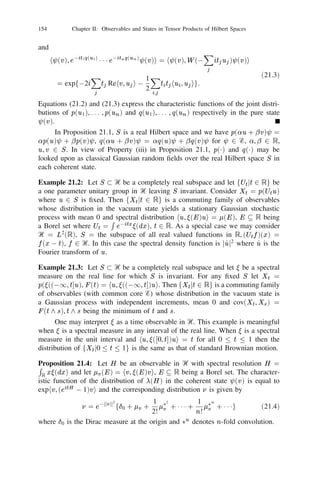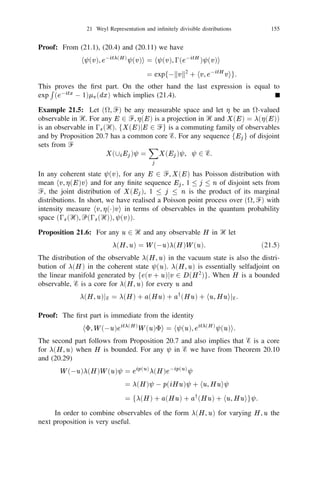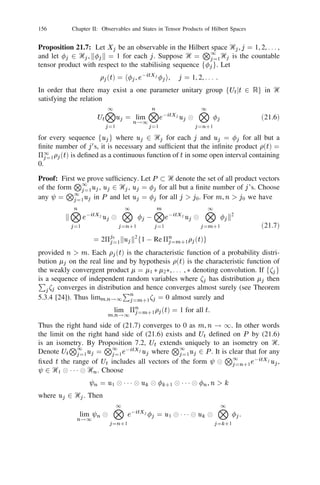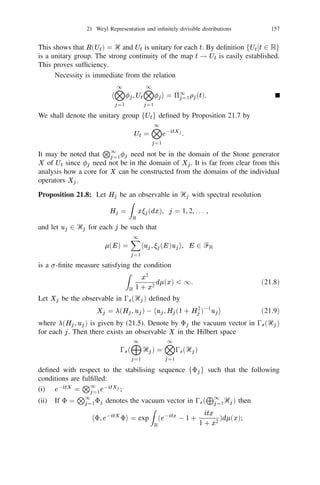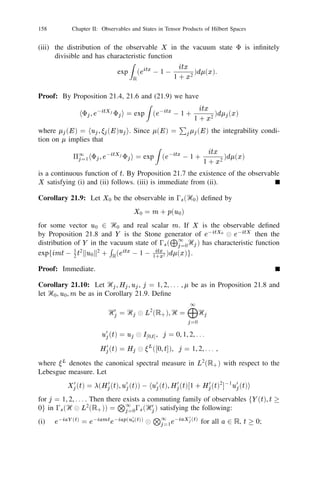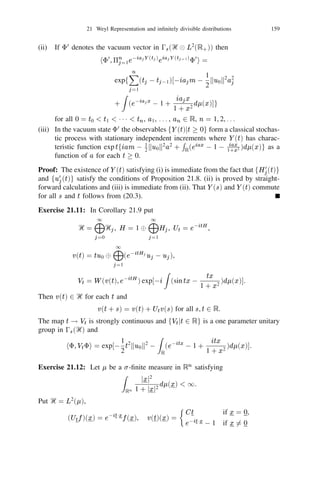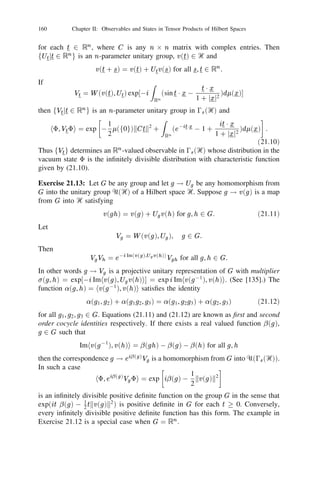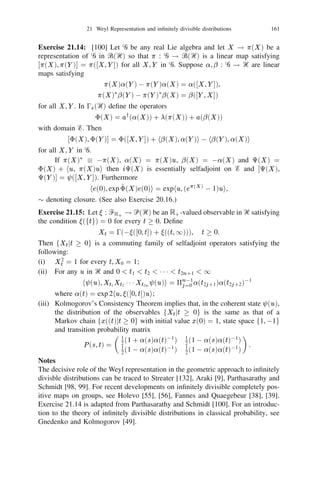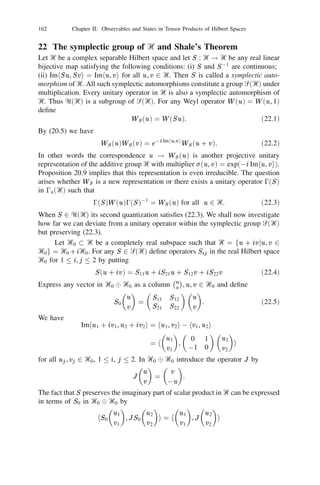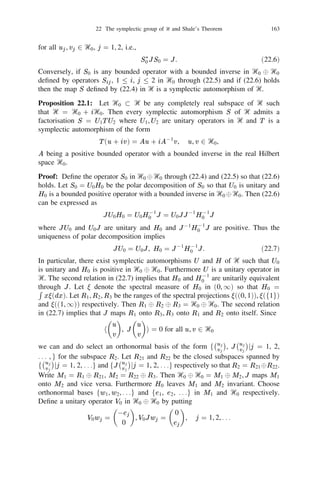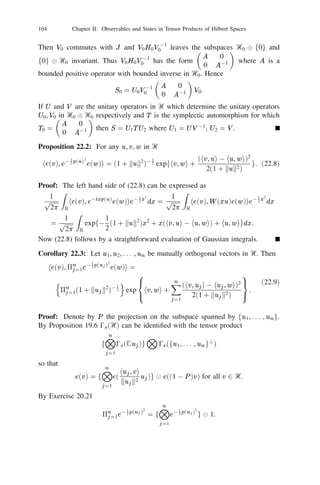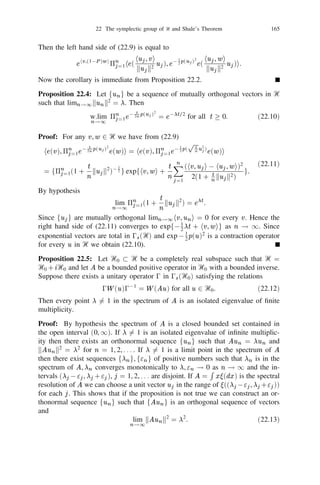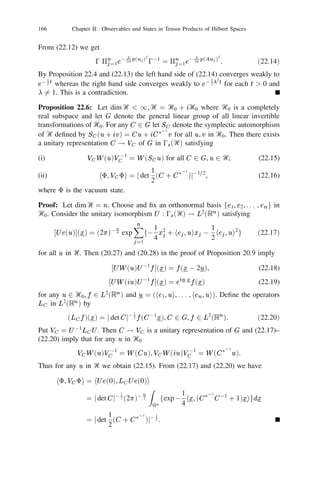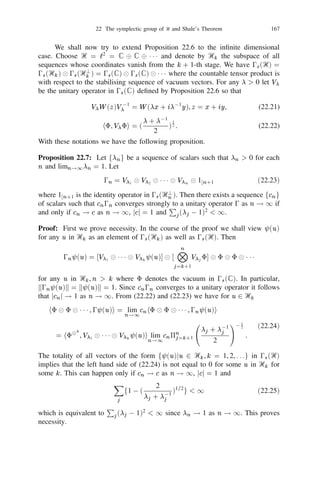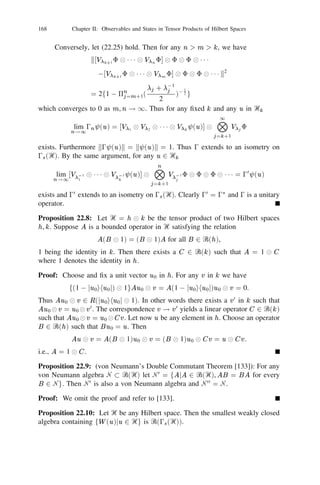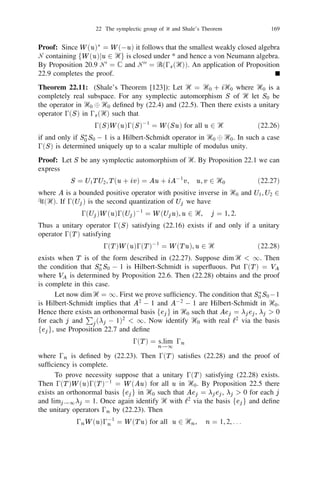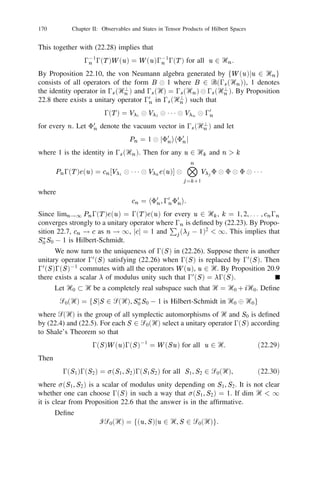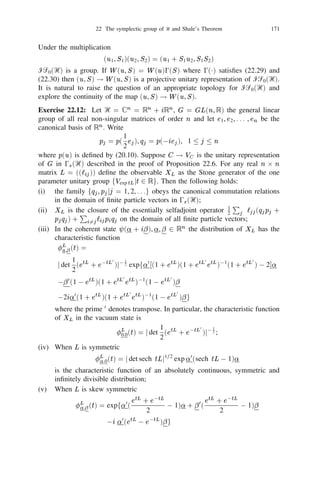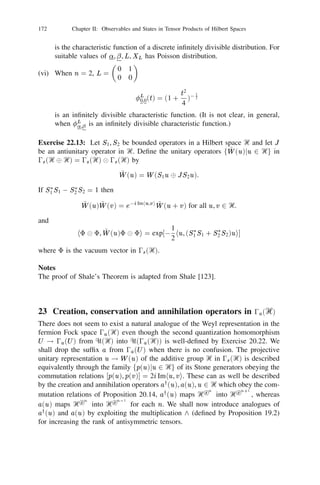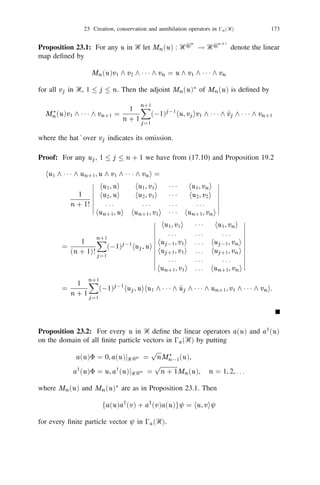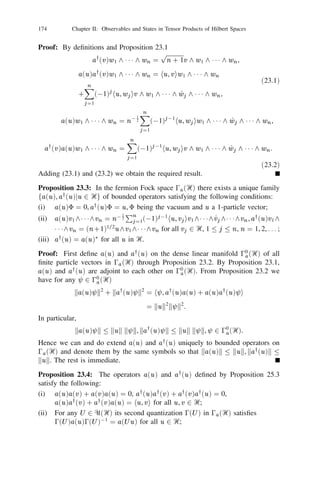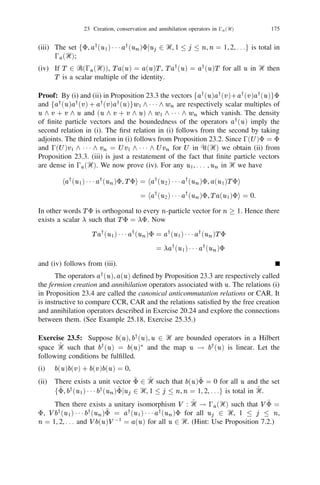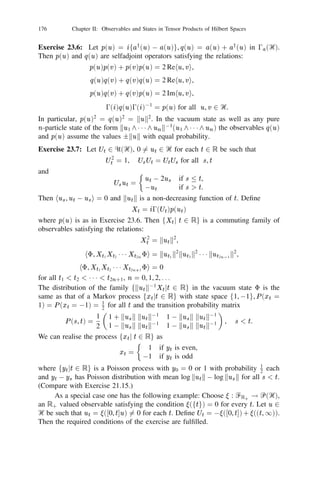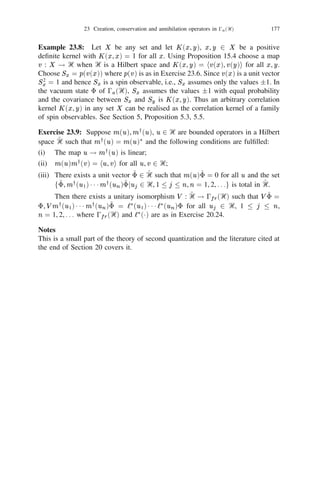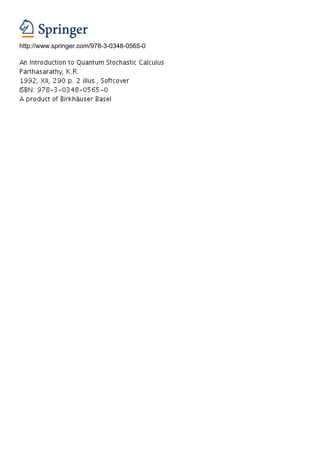Nächste SlideShare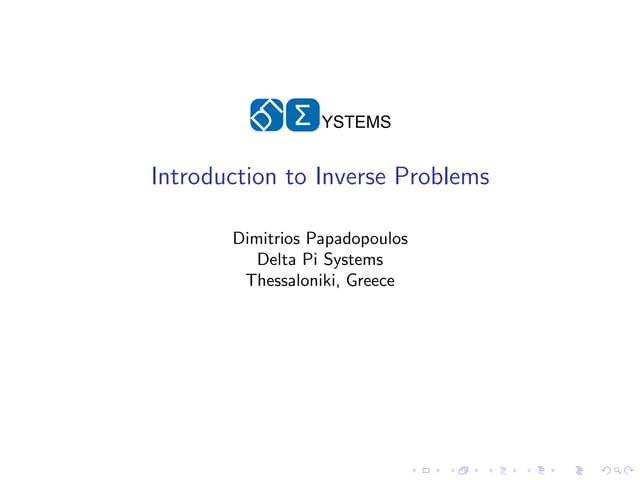Introduction to inverse problems
1 von 88
Anzeige

### An introduction to quantum stochastic calculus

1. Chapter II Observables and States in Tensor Products of Hilbert Spaces 15 Positive deﬁnite kernels and tensor products of Hilbert spaces Suppose ( i , Ᏺi ), 1 i n are sample spaces describing the elementary out- comes and events concerning n different statistical systems in classical proba- bility. To integrate them into a uniﬁed picture under the umbrella of a single sample space one takes their cartesian product ( , Ᏺ) where = 1 2 1 1 1 2 n , Ᏺ = Ᏺ1 2 1 1 1 2 Ᏺn , the smallest -algebra containing all rectangles of the form F1 2 F2 21 1 12 Fn , Fj 2 Ᏺj for each j . Now we wish to search for an analogue of this description in quantum probability when we have n systems where the events concerning the j -th system are described by the set P (Ᏼj ) of all projections in a Hilbert space Ᏼj , j = 1, 2, . . . , n. Such an attempt leads us to consider tensor products of Hilbert spaces. We shall present a somewhat statistically oriented ap- proach to the deﬁnition of tensor products which is at the same time coordinate free in character. To this end we introduce the notion of a positive deﬁnite kernel. X Let ᐄ be any set and let K : ᐄ 2 ᐄ ! ‫ ރ‬be a map satisfying the following: i j K (xi , xj ) 0 i,j for all i 2 ‫ ,ރ‬xi 2 ᐄ, i = 1, 2, . . . , n. Such a map K is called a positive deﬁnite kernel or simply a kernel on ᐄ. We denote by K(ᐄ) the set of all such kernels on ᐄ. If ᐄ = f1, 2, . . . , ng, a kernel on ᐄ is just a positive (semi) deﬁnite matrix. If Ᏼ is a Hilbert space the scalar product K (x, y ) = hx, y i is a kernel on Ᏼ. If G is a group and g ! Ug is a homomorphism from G into the unitary group ᐁ(Ᏼ) of a Hilbert space Ᏼ then K (g , h) = hu, Ug01 h ui is a kernel on G for every u in Ᏼ. If is a state in Ᏼ then K (X , Y ) = tr X 3 Y is a kernel on Ꮾ(Ᏼ). Proposition 15.1: Let ((aij )), ((bij )), 1 i, j n be two positive deﬁnite matrices. Then ((aij bij )) is positive deﬁnite. Proof: Let A = ((aij )). Choose any matrix C of order n such that CC 3 = A. Let 1 , . . . , n ,
2. 1 , . . . ,
3. n be any 2n independent and identically distributed standard Gaussian (normal) random variables. Write j = 20 2 (j + i
4. j ), = C where 1 denotes the column vector with j -th entry j . Then is a complex valued Gaussian random vector satisfying ‫ = 3 ޅ ,0 = ޅ‬CC 3 = A. Using the procedure described above select a pair of independent complex Gaussian random vectors , such that ‫ޅ‬j = ‫ޅ‬j = 0, ‫ ޅ‬i j = aij , ‫ ޅ‬i j = bij K.R. Parthasarathy, An Introduction to Quantum Stochastic Calculus, 91 DOI 10.1007/978-3-0348-0566-7_2, © Springer Basel 1992
5. 92 Chapter II: Observables and States in Tensor Products of Hilbert Spaces for 1 i, j n. Let j = j j . Then ‫ޅ‬j = 0, ‫ ޅ‬i j = aij bij . Thus ((aij bij )) is the covariance matrix of the complex random vector and hence positive deﬁnite. Corollary 15.2: The space K (ᐄ) of all kernels on ᐄ is closed under pointwise multiplication. Proof: This follows immediately from Proposition 15.1 and the fact that K is a kernel on ᐄ if and only if for any ﬁnite set fx1 , x2 , . . . , xn g ᐄ the matrix ((aij )) where aij = K (xi , xj ) is positive deﬁnite. Corollary 15.3: Let ᐄi , 1 i n be sets and let Ki 2 K (ᐄ ) for each i. Deﬁne i ᐄ = ᐄ1 2 1 1 1 2 ᐄn and ( ) = 5 =1 K (x , y ), x = (x1 , . . . , x ), y = (y1 , . . . , y ) K x, y n i i i i n n where x , y 2 ᐄ . Then K 2 K (ᐄ). i i i Proof: Let x( ) = (x1 , x2 , . . . , x ) 2 ᐄ, 1 r m. Putting a = r r r nr i rs K (x , x ) we observe that i ir is K (x , x ) = 5 =1 a . ( ) ( ) r s n i i rs Since ((a )), 1 r, s m is positive deﬁnite for each ﬁxed i the required result i rs follows from Proposition 15.1. Proposition 15.4: Let ᐄ be any set and let K 2 K (ᐄ). Then there exists a (not necessarily separable) Hilbert space Ᏼ and a map : ᐄ ! Ᏼ satisfying the following: (i) the set f(x), x 2 ᐄg is total in Ᏼ; (ii) K (x, y ) = h(x), (y )i for all x, y in ᐄ. If Ᏼ0 is another Hilbert space and 0 : ᐄ ! Ᏼ0 is another map satisfy- ing (i) and (ii) with Ᏼ, replaced by Ᏼ0 , 0 respectively then there is a unitary isomorphism U : Ᏼ ! Ᏼ0 such that U (x) = 0 (x) for all x in ᐄ. Proof: For any ﬁnite set F = fx1 , x2 , . . . , xn g ᐄ it follows from the argument in the proof of Proposition 15.1 that there exists a complex Gaussian random vector (1 , 2 , . . . , n ) such that F F F = K (x , x ), ‫ 1 ,0 = ޅ‬i, j n. F F ‫ޅ‬i j i j F i If G = fx1 , x2 , . . . , x , x +1 g F then the marginal distribution of (1 , . . . , ) n n G G n derived from that of (1 , . . . , , +1 ) is the same as the distribution of (1 , G G n G F . . . , ). Hence by Kolmogorov’s Consistency Theorem there exists a Gaussian n F n family f , x 2 ᐄg of complex valued random variables on a probability space x ( , Ᏺ, P ) such that ‫ = ޅ ,0 = ޅ‬K (x, y ) for all x, y 2 ᐄ. x x y If Ᏼ is the closed linear span of f , x 2 ᐄg in L2 (P ) and (x) = then the x x ﬁrst part of the proposition holds.
6. 15 Positive deﬁnite kernels and tensor products of Hilbert spaces 93 To prove the second part consider S = f(x)jx 2 ᐄg, S 0 = f0 (x)jx 2 ᐄg and the map U : (x) ! 0 (x) from S onto S 0 . Then U is scalar product preserving and S and S 0 are total in Ᏼ and Ᏼ0 respectively. By the obvious generalisation of Proposition 7.2 for not necessarily separable Hilbert spaces, U extends uniquely to a unitary isomorphism from Ᏼ onto Ᏼ0 . The pair (Ᏼ, ) determined uniquely up to a unitary isomorphism by the kernel K on ᐄ is called a Gelfand pair associated with K . We are now ready to introduce the notion of tensor products of Hilbert spaces using Proposition 15.4. Let Ᏼi , 1 i n be Hilbert spaces and let ᐄ = Ᏼ1 , 2 1 1 1 2 Ᏼn be their cartesian product as a set. Then the function Ki (u, v ) = hu, v i, u, v 2 Ᏼi is a kernel on Ᏼi for each i. By Corollary 15.3 the function K (u, v ) = 5n=1 hui , vi i, u = (u1 , . . . , un ), v = (v1 , . . . , vn ) i where ui , vi 2 Ᏼi for each i, is a kernel on ᐄ. Consider any Gelfand pair (Ᏼ, ) associated with K and satisfying (i) and (ii) of Proposition 15.4. Then Ᏼ is called a tensor product of Ᏼi , i = 1, 2, . . . , n. We write Ᏼ = Ᏼ1 Ᏼ2 1 1 1 Ᏼn O = n Ᏼi , (15.1) O = i 1 n (u) = u1 u2 1 1 1 u = u n i (15.2) i =1 and call (u) the tensor product of the vectors ui , 1 i n. If Ᏼi = h for all i then Ᏼ is called the n-fold tensor product of h and denoted by h . If, in addition, n ui = u for all i in (15.2) then (u) is denoted by u and called the n-th power n of u. (Since (Ᏼ, ) is determined uniquely upto a Hilbert space isomorphism we take the liberty of calling Ᏼ the tensor product of Ᏼi , 1 i n in (15.1)). Proposition 15.5: The map (u1 , u2 , . . . , un ) ! u1 u2 1 1 1 un from Ᏼ1 2 Ᏼ2 2 1 1 1 2 Ᏼn into Ᏼ1 Ᏼ2 1 1 1 Ᏼn deﬁned by (15.1) and (15.2) is multilinear: for all scalars ,
7. u1 1 1 1 ui01 (ui +
8. vi ) ui+1 1 1 1 un (15.3) = u1 1 1 1 u +
9. u1 1 1 1 u 01 v n i i ui+1 1 1 1 un (15.4) Furthermore h Ou Ov n , n i = 5n 1 hui , vi i. i= (15.5) N= u u N= i i =1 i =1 i 2 Ᏼi , i = 1, 2, . . . , ng is total in n n The set f i 1 ij i i 1 Ᏼi .
10. 94 Chapter II: Observables and States in Tensor Products of Hilbert Spaces Proof: Only (15.3) remains to be proved. Straightforward computation using O (15.4) and the sesquilinearity of scalar products show that for each ﬁxed i n ku1 1 1 1 ui01 (ui +
11. vi ) ui+1 1 1 1 un 0 uj j =1 0
12. u1 1 1 1 ui01 vi ui+1 1 1 1 un k2 = 5j 6=i kuj k fkui +
13. vi k2 + jj2 kui k2 + j
14. j2 kvi k2 2 02 Re(hui +
15. vi , ui i + hui +
16. vi ,
17. vi i + hui ,
18. vi i)g N= = 0. n For any ui 2 Ᏼi , 1 i n the product vector i 1 ui may be interpreted O as the multi-antilinear functional n ( ui )(v1 , v2 , . . . , vn ) = 5n 1 hvi , ui i. i= =1 i Such multi-antilinear functionals generate a linear manifold M to which the scalar N product (15.4) can be extended by sesquilinearity to make it a pre-Hilbert space. M is the usual algebraic tensor product of the vector spaces Ᏼi , 1 i n and n i=1 Ᏼi is its completion. Exercise 15.6: (i) Let K be a kernel on ᐄ. A bijective map g : ᐄ ! ᐄ is said to leave K invariant if K (g (x), g (y )) = K (x, y ) for all x, y in ᐄ. Let GK denote the group of all such bijective transformations of ᐄ leaving K invariant and let (Ᏼ, ) be a Gelfand pair associated with K . Then there exists a unique homomorphism g ! Ug from GK into the unitary group ᐁ(Ᏼ) of Ᏼ satisfying the relation Ug (x) = (g (x)) for all x 2 ᐄ, g 2 GK . If (Ᏼ0 , 0 ) is another Gelfand pair associated with K , V : Ᏼ ! Ᏼ0 is the unitary isomorphism satisfying V (x) = 0 (x) for all x and g ! Ug is the homomor- 0 phism from GK into U (Ᏼ0 ) satisfying Ug 0 (x) = 0 (g (x)) for all x 2 ᐄ, g 2 GK 0 then V Ug V 01 = Ug for all g . 0 (ii) In (i) let ᐄ be a separable metric space and let K be continuous on ᐄ 2 ᐄ. Suppose G0 GK is a subgroup which is a topological group acting continuously on ᐄ 2 ᐄ. Then in any Gelfand pair (Ᏼ, ) the map : ᐄ ! Ᏼ is continuous, Ᏼ is separable and the homomorphism g ! Ug restricted to G0 is continuous. (Hint: Use Proposition 7.2 for (i) and examine k(x) 0 (y )k for (ii)). n N Exercise 15.7: (i) Let Ᏼi , 1 i n be Hilbert spaces and let Si Ᏼi be a total n subset for each i. Then the set f i=1 ui jui 2 Si for each ig is total in i=1 Ᏼi . N Ᏼ is separable if each Ᏼi is separable.
19. 15 Positive deﬁnite kernels and tensor products of Hilbert spaces 95 (ii) There exist unitary isomorphisms U12,3 : (Ᏼ1 Ᏼ2 ) Ᏼ3 ! Ᏼ1 Ᏼ2 Ᏼ3 , U1,23 : Ᏼ1 (Ᏼ2 Ᏼ3 ) ! Ᏼ1 Ᏼ2 Ᏼ3 , such that U12,3 (u1 u2 ) u3 = U1,23 u1 (u2 u3 ) = u1 u2 u3 for all i 2 Ᏼi , i = 1, 2, 3. (Hint: Use Proposition 7.2.) Exercise 15.8: Let feij jj = 1, 2, . . .g be an orthonormal basis in Ᏼi , i = 1, 2, . . . , n respectively. Then the set fe1j e2j 1 1 1 enjn jj1 = 1, 2, . . . , j2 = 1, 2, . . . , jn = 1, 2, . . .g 1 2 N is an orthonormal basis for i=1 Ᏼi . (Note that when dim Ᏼi = mi 1, ji = n 1, 2, . . . , mi ). In particular, Ou n i = X f n 5i=1 he iji , u ig i Oe n iji =1 i j1 ,j2 ,... ,jn where the right hand side is a strongly convergent sum in i=1 N n Ᏼi . If dim Ᏼi = mi 1 for every i then i=1 dim OᏴn i = m1 m2 1 1 1 mn . i=1 Exercise 15.9: Let Ᏼi = L2 ( i , Ᏺi , i ), 1 i n where ( i , Ᏺi , i ) is a -ﬁnite measure space for each i. If ( , Ᏺ, ) = 5n 1 ( i , Ᏺi , i ) is the cartesian product i= of these measure spaces there exists a unitary isomorphism U : n i=1 Ᏼi ! N L ( , Ᏺ, ) such that 2 (Uu1 1 1 1 un )(!1 , . . . , !n ) = 5n 1 ui (!i ) a.e. . i = Exercise 15.10: Let Ᏼn , n = 1, 2, . . . be a sequence of Hilbert spaces and let f g be a sequence of unit vectors where 2 Ᏼ for each n. Suppose n n n M = fuju = (u1 , u2 , . . .), u 2 Ᏼ , u = for all large ng. j j n n K (u, v) = 51 1 huj , vj i, u, v 2 M . Deﬁne j = Then K is a kernel on M . The Hilbert space Ᏼ in a Gelfand pair (Ᏼ, ) associated with K is called the countable tensor product of the sequence fᏴn g with respect to the stabilizing sequence fn g. We write (u) = u1 u2 1 1 1 for u 2 M . Suppose fen0 , en1 , . . .g = Sn is an orthonormal basis in Ᏼn such that en0 = n for each n. Then the set f(u)ju 2 M , uj 2 Sj for each j g is an orthonormal basis in Ᏼ.
20. 96 Chapter II: Observables and States in Tensor Products of Hilbert Spaces Exercise 15.11: Let Ᏼn = L2 ( n , Ᏺn , n ), n = 1, 2, . . . , Ᏼ = L2 ( , Ᏺ, ) where ( n , Ᏺn , n ) is a probability space for each n and ( , Ᏺ, ) = 51 1 ( n , n= Ᏺn , n ) is the product probability space. Then Ᏼ is the countable tensor product of the sequence fᏴn g with respect to the stabilising sequence fn g where n is the constant function 1 in n for each n. This may be used to construct examples where different stabilising sequences may lead to different countable tensor prod- ucts. (Hint: If and are two distinct probability measures such that then 2 2 1 1 1 and 2 2 1 1 1 are singular with respect to each other.) Exerecise 15.12: Let Ᏼ = L2 ([0, 1], h) be the Hilbert space deﬁned in Section 2 where is the Lebesgue measure in [0, 1]. Then any continuous map f : [0, 1] ! Ᏼ is an element of Ᏼ. For any such continuous map f deﬁne the element en (f ) in (‫ 8 ރ‬h) n by en (f ) = n=1 f1 8 n0 21 f ( j )g. j n Then lim hen (f ), en (g )i = exphf , g i. n !1 Exercise 15.13: Let Ᏼ1 , Ᏼ2 be Hilbert spaces and let ff1 , f2 , . . .g be an or- thonormal basis in Ᏼ2 . Then there exists a unitary isomorphism U : Ᏼ1 Ᏼ2 ! Ᏼ1 8 Ᏼ1 8 1 1 1 satisfying Uu v = 8i hfi , v iu for all u 2 Ᏼ1 , v 2 Ᏼ2 . Exercise 15.14: Let L2 (, h) be as deﬁned in Section 2. Then there exists a unique unitary isomorphism U : L2 () h ! L2 (, h) satisfying (Uf u)(! ) = f (! )u. Exercise 15.15: Let Ᏼ be a Hilbert space and let I2 (Ᏼ) be the Hilbert space of 3 all Hilbert-Schmidt operators in Ᏼ with scalar product hT1 , T2 i = tr T1 T2 . For any ﬁxed conjugation J there exists a unique unitary isomorphism U : Ᏼ Ᏼ ! I2 (Ᏼ) satisfying Uu v = juihJv j for all u, v in Ᏼ. (See Section 9.) Example 15.16:  Let H be any selfadjoint operator in a Hilbert space Ᏼ with pure point spectrum 6(H ) = S . Then S is a ﬁnite or countable subset of ‫ .ޒ‬Denote by G the countable additive group generated by S and endowed with ~ the discrete topology. Let G be its compact character group with the normalised Haar measure. For any bounded operator X on Ᏼ and 2 G deﬁne the bounded operator Z X = ()(H )X(H )d. ~ G Let , 2 S , u, v 2 Ᏼ, Hu = u, Hv = v. Then Z hu, X v i = f ( 0 0 )dghu, Xvi. ~ G Hence hu, Xvi = 0 , hu, X v i = if 0 if 6= 0 .
21. 16 Operators in tensor products of Hilbert spaces 97 Thus, for any non-zero bounded operator X there exists a 2 S0S = S X f 0 j , 2 g such that 6= 0 and on the linear manifold D generated by all the eigenvectors of H [H , [H , X ]] = 2 X , Xu = X0u + X (X0 + X )u , u 2 D. 0 2S0S IfX X X is selfadjoint ( )3 = 0 and X is a “superposition” of bounded “har- X X X S S monic” observables 0 , f 0 + j 2 0 , 0g with respect to . (See H XY tr X 3 Y = tr X0 Y0 + 3 X Example 6.2.) Whenever , are Hilbert-Schmidt operators tr X Y 3 2S0S X (X + X 0 and X = X0 + 0 ) 2S0S 0 converges in Hilbert-Schmidt norm (i.e., the norm in I2 (Ᏼ)). See Example 6.2. Notes The presentation here is based on the notes of Parthasarathy and Schmidt . Proposition 15.1 is known as Schur’s Lemma. Proposition 15.4 and Exercise 15.6 constitute what may be called a probability theorist’s translation of the famous Gelfand-Neumark-Segal or G.N.S. Theorem. Its origin may be traced back to the theory of second order stationary stochastic processes developed by A.N. Kol- mogorov, N. Wiener, A.I. Khinchine and K. Karhunen . Proposition 7.2, 15.4 and 19.4 more or less constitute the basic principles around which the fabric of our exposition in this volume is woven. e n h It is an interesting idea of Journ´ and Meyer  that (‫ ) 8 ރ‬in Exercise e f 15.12 may be looked upon as a toy Fock space where n ( ) may be imagined as a toy exponential or coherent vector which in the limit becomes the boson Fock L h ef space 0( 2 [0, 1] ) where ( ) is the true exponential or coherent vector. See Exercise 29.12, 29.13, Parthasarathy , Lindsay and Parthasarathy . 16 Operators in tensor products of Hilbert spaces n N We shall now deﬁne tensor products of operators. To begin with let Ᏼi be a ﬁnite m i dimensional Hilbert space of dimension i , = 1, 2, . . . , and Ᏼ = i=1 Ᏼi . n T j m Suppose i is a selfadjoint operator in Ᏼi with eigenvalues f ij , 1 i g e j m and corresponding orthnormal set of eigenvectors f ij , 1 i g so that Tieij = ij eij , 1 j mi, i = 1, 2, . . . , n. Using Exercise 15.8 deﬁne a selfadjoint operator T on Ᏼ by putting On On T eiji = 5n=1iji eiji , 1 ji mi i i=1 i=1
22. 98 Chapter II: Observables and States in Tensor Products of Hilbert Spaces T O =O and extending linearly on Ᏼ. The operator T has eigenvalues 5n 1 iji and satisﬁes n ui i= n Ti ui for all ui 2 Ᏼi , 1 i n. =1 i =1 i Furthermore kT k = max (j5n=1 ij j, 1 ji mi ) i i = 5i=1 max(jij j, 1 j mi ) = 5n=1 kTi k. n i X XO XO In particular, we have the identity N n N n j aj k 5 =1 huij , Ti uikn i ij = jh j uij , T j uij ij 1 j ,k N = j = 5= k kk n i XO k 1 = 1 = Ti i 1 N j j 1 n i uij 2 1 (16.1) =1 =1 N= j i 2 Ᏼi , 1 j N , 1 i n, N = 1, 2, . . . . We write for all scalars j , uij T = 1 Ti = T1 1 1 1 Tn and call it the tensor product of operators Ti , n i 1 i n. The next proposition extends this elementary notion to all bounded operators on Hilbert spaces. Proposition 16.1: Let Ᏼi , 1 i n be Hilbert spaces and let Ti be a bounded N operator in Ᏼi for each i. Then there exists a unique bounded operator T in Ᏼ = i=1 Ᏼi satisfying O =O n n n T 2 ui Ti ui for all ui Ᏼi , 1 i n. (16.2) = = = fN = j 2 i 1 i 1 Furthermore T k k=5k k Ti . N = 2 g i Proof: Let S n i 1 i u ui Ᏼi , 1 i n . For all scalars j , 1 j N 1 uij S , 1 j N we have n X O k = X 5= h and product vectors i N n k j Ti uij j k n uij , Ti3 Ti uik i X 5= h 2 i 1 j= 1 = i 1 1 j ,k N = j k n i 1 uij , Pi Ti3 Ti Pi uik i (16.3) 1 j ,k N where Pi is the projection on the ﬁnite dimensional subspace Mi spanned by fuij , 1 j N g in Ᏼi for each i. Since Pi Ti3 Ti Pi is a positive operator in Mi , k XO it follows from (16.1) and (16.3) that N j n Ti uij k2 5n=1 kPi Ti3 Ti Pi k k i XO N j n uij k2 j =1 i =1 5n=1 kTi3 Ti i kk XO k = = N j j 1 n i uij 2 . 1 (16.4) j =1 i=1
23. 16 Operators in tensor products of Hilbert spaces 99 Deﬁne T on the set S by (16.2). Then (16.4) shows that T extends uniquely as a linear map on the linear manifold generated by S . Since S is total in Ᏼ this linear extension can be closed to deﬁne a bounded operator T on Ᏼ satisfying kT k 5n kT 3 T k1=2 = 5n kT k.=1 i i i =1 i i (1 0 )kT k for each i, assuming T 6= 0. Then N =1 u Let now 0 1 be arbitrary. Choose unit vectors ui 2 Ᏼi such that kTi ui k n i i i is a unit vector in Ᏼ and Ou k = k OT u k = 5 i n n kT =1 kTi ui k (1 0 ) 5 =1 k T k . n n n i i i i i i =1 i i=1 Thus kT k 5i=1 kTi k. n The operator T determined by Proposition 16.1 is called the tensor productN of the operators Ti , 1 i n. We write T = i=1 Ti = T1 1 1 1 Tn . If Ᏼi = h, n Ti = S for all i = 1, 2, . . . , n we write T = S and call it the n-th tensor power n of the operator S . N Proposition 16.2: Let Ᏼi , 1 i n be Hilbert spaces and let Si , Ti be bounded operators in Ᏼi for each i. Let S = i=1 Si , T = i=1 Ti . Then the following n n N relations hold: (i) The mapping (T1 , T2 , . . . , Tn ) ! T is multilinear from B (Ᏼ1 ) 21 1 12B (Ᏼn ) into B (Ᏼ1 1 1 1 Ᏼn ); N (ii) ST = i=1 Si Ti , T 3 = i=1 Ti3 ; n n N (iii) If each Ti has a bounded inverse then T has a bounded inverse and T 01 = i Ti01 ; (iv) T is a selfadjoint, unitary, normal or projection operator according to whether each Ti is a selfadjoint, unitary, normal or projection operator; (v) T is positive if each Ti is positive; (vi) If Ti = ju ihv j where u , v 2 Ᏼ for each i then i i i i i T = ju1 u2 1 1 1 u ihv1 v2 1 1 1 v n n j. Proof: This is straightforward from deﬁnitions and we omit the proof. N n i=1 n N Proposition 16.3: Let Ti be a compact operator in Ᏼi , i = 1, 2, . . . , n, Ᏼ = Ᏼi , T = T . If Ti has the canonical decomposition in the sense of i=1 i Proposition 9.6 Ti = X s (T )jv j i ij ihuij j, i = 1, 2, . . . , n (16.5) j then T is a compact operator with canonical decomposition T = X sj1 (T1 ) 1 1 1 sjn (Tn )jv1j1 1 1 1 vnjn ihu1j1 1 1 1 unjn j. (16.6) j1 ,j2 ,... ,jn
24. 100 Chapter II: Observables and States in Tensor Products of Hilbert Spaces If Ti 2 I1 (Ᏼi ) for each i then T 2 I1 (Ᏼ) and kT k1 = 5n=1 kTi k1 , tr T i = 5i=1 tr Ti . n In particular, if each Ti is a state so is T . Proof: Since fuij , j = 1, 2, . . .g, fvij , j = 1, 2, . . .g are orthonormal sets in Ᏼi for each i it follows that fu1j1 1 1 1 unjn g, fv1j1 1 1 1 vnjn g are orthonormal sets in Ᏼ. Equation (16.5), the second part of Proposition 16.1 and (vi) in Proposition 16.2 P yield (16.6) as an operator norm convergent sum over the indices (j1 , j2 , . . . , jn ). X If kTi k1 = j jsj (Ti )j 1 for each i then we have kT k 1 = jsj (T1 ) 1 1 1 sjn (Tn )j = 5n 1 kTi k1 . 1 i = j1 ,j2 ,... ,jn If feij , j = 1, 2, . . .g is an orthonormal basis in Ᏼi for each i it follows that fe1j 1 1 1 enjn g X he 1 1 1 e T 1 1 1 T e 1 is an orthonormal basis in Ᏼ and tr T = njn , 1 1 1 enjn i X he T e i 1j1 1 n 1j1 j1 ,... ,jn n = 5i=1 , Xhe T e i iji i iji j1 ,... ,jn n = 5i=1 ij , i ij j = 5i=1 tr Ti , n due to the absolute convergence of all the sums involved. Finally, if each Ti is positive so is T . If tr Ti = 1 for each i then tr T = 1. then putting Ᏼ = n i=1 N n i=1 i N If (Ᏼi , P (Ᏼi ), i ) is a quantum probability space for each i = 1, 2, . . . , n Ᏼi , = we obtain a new quantum probability space (Ᏼ, P (Ᏼ), ) called the product of the quantum probability spaces (Ᏼi , P (Ᏼi ), i ), i = 1, 2, . . . , n. If Xi is a bounded selfadjoint operator in Ᏼi or, equivalently, a bounded real valued observable in Ᏼi then X = X1 1 1 1 Xn is a bounded real valued observable in Ᏼ and the expectation of X in the product state is equal to 5n 1 tr i Xi , the product of the expectations of Xi in the state i , i = 1, 2, . . . , n. i= Now the stage is set for achieving our goal of combining the description of events concerning several statistical experiments into those of a single experiment. Suppose that the events of the i-th experiment are described by the elements of P (Ᏼi ) where Ᏼi is a Hilbert space, i = 1, 2, . . . , n. Let Ᏼ = i Ᏼi . If Pi 2 P (Ᏼi ) then we view the event Pi as the element Pi = 1 1 1 1 1 Pi 1 1 1 1, ^
25. 16 Operators in tensor products of Hilbert spaces 101 (called the ampliation of Pi in Ᏼ) in ᏼ(Ᏼ) and interpret P = i=1 Pi = 5n 1 Pi 2i= ^ N n ᏼ(Ᏼ) (see Proposition 16.2) as the event signifying the simultaneous occurrence of Pi in the i-th experiment for i = 1, 2, . . . , n. These are the quantum probabilis- tic equivalents of measurable rectangles in products of sample spaces. The next proposition indicates how ᏼ(Ᏼ) is “generated” by P (Ᏼi ), i = 1, 2, . . . , n. Proposition 16.4: Every element P in ᏼ(Ᏼ) can be obtained as a strong limit of linear combinations of projections of the form i=1 Pi , Pi 2 P (Ᏼi ). n N Proof: Choose an increasing sequence fQik , k = 1, 2, . . .g of ﬁnite dimen- N sional projections so that s.limk!1 Qik = 1 in Ᏼi for each i. Then Qk = n Q increases to the identity strongly in Ᏼ and for any bounded opera- i=1 ik tor T in Ᏼ, s.limK !1 Qk T Qk = T . Thus it sufﬁces to show that for ﬁxed k the N operator Qk T Qk can be expressed as a linear combination of product projections of the form i=1 Pi , Pi 2 P (Ᏼi ). Replacing Ᏼi by the range of Qik if necessary n we may assume that each Ᏼi has dimension mi 1. Let feir , 1 r mi g be an orthonormal basis in Ᏼi and let Ers = jeis iheir j. Then every operator T on i Ᏼ can be expressed as a linear combination of operators of the form i=1 Erj sj . n j N Each Ers can be expressed as a linear combination of selfadjoint operators: j j3 1 j 1 j 3 Ers = (Ers + Ers ) + if (Ers 0 Ers )g. j j 2 2i Since every selfadjoint operator in Ᏼi admits a spectral decomposition in terms of eigen projections the proof is complete. Proposition 16.5: Let ( i , Ᏺi ) be measurable spaces and let i : Ᏺi ! P (Hi ) n i= be the product measurable space and Ᏼ = i=1 Ᏼi . Then there exists a unique N be an i -valued observable in Ᏼi for i = 1, 2, . . . , n. Let ( , Ᏺ) = 5n 1 ( i , Ᏺi ) -valued observable : Ᏺ ! ᏼ(Ᏼ) such that (F1 2 1 1 1 2 Fn ) = O n Fi ) for all Fi 2 Ᏺi , i = 1, 2, . . . , n. i( (16.7) =1 i Proof: By Proposition 7.4 there exists a ﬁnite or countable family of -ﬁnite measures fij , j = 1, 2, . . . , g and a unitary isomorphism Ui : Ᏼi ! j L2 (ij ) L such that Ui i (E )Ui01 = M ij E ) for all E 2 Ᏺi , ( 1 i n. j Of8 L ML We have n j 2 ij )g = ( 2 2 2j 2 1 1 1 2 njn ). ( 1j 1 2 =1i j1 ,... ,jn Deﬁne U = U1 1 1 1 Un and (F ) = U 01 f M 1j1 21112 njn F gU , F 2 Ᏺ. ( ) j1 ,... ,jn Then (16.7) is obtained.
26. 102 Chapter II: Observables and States in Tensor Products of Hilbert Spaces The unique observable satisfying (16.7) in Proposition 16.5 is called the tensor product of the observables i , i = 1, 2, . . . , n. We write = 1 1 1 1 n . Proposition 16.6: Let Ᏼ1 , Ᏼ2 be Hilbert spaces and let T be a trace class operator in Ᏼ = Ᏼ1 Ᏼ2 . Then there exists a unique trace class operator T1 in Ᏼ1 such that tr T1 X = tr T (X 1) for all X in B(Ᏼ1 ). (16.8) If T is a state in Ᏼ then T1 is a state in Ᏼ1 . Proof: For any compact operator X in Ᏼ1 deﬁne (X ) = tr T (X 1). By Proposition 9.12, (iii) we have j(X )j kT k1 kX k. In particular, is a continuous linear functional on I1 (Ᏼ1 ). By Schatten’s Theorem (Theorem 9.17) there exists a T1 in I1 (Ᏼ1 ) such that tr T1 X = tr T (X 1) for all X in I1 (Ᏼ1 ). Since the maps X ! tr T1 X and X ! tr T (X 1) are strongly continuous in B(Ᏼ1 ) we obtain (16.8). If T is positive we have hu, T1 ui = tr T1 juihuj = tr T (juihuj 1) 0 for all u in Ᏼ1 . Thus T1 0. Putting X = 1 in (16.8) we get tr T1 = tr T . This proves that T1 is a state if T is a state. The operator T1 in Proposition 16.6 is called the relative trace of T in Ᏼ1 . If T is a state then the relative trace of T1 is the analogue of marginal distribution in classical probability. Exercise 16.7: Suppose Ᏼ1 , Ᏼ2 are two real ﬁnite dimensional Hilbert spaces of dimensions m1 , m2 respectively and Ᏼ = Ᏼ1 Ᏼ2 . Let ᏻ(Ᏼ1 ), ᏻ(Ᏼ2 ) and ᏻ(Ᏼ1 Ᏼ2 ) be the real linear spaces of all selfadjoint operators in Ᏼ1 , Ᏼ2 and Ᏼ1 Ᏼ2 respectively. Then we have dim ᏻ(Ᏼi ) = 2 mi (mi + 1), i = 1, 2 and 1 dim ᏻ(Ᏼ1 Ᏼ2 ) = 2 m1 m2 (m1 m2 + 1). In particular, 1 dim ᏻ(Ᏼ1 Ᏼ2 ) dim ᏻ(Ᏼ1 ) 1 dim ᏻ(Ᏼ2 ) if mi 1, i = 1, 2, . On the other hand, if Ᏼ1 , Ᏼ2 are complex Hilbert spaces dim ᏻ(Ᏼi ) = mi and 2 dim ᏻ(Ᏼ1 Ᏼ2 ) = dim ᏻ(Ᏼ1 ) dim ᏻ(Ᏼ2 ). (In the light of Proposition 16.4 this indicates the advantage of working with complex Hilbert spaces in dealing with observables concerning several quantum statistical experiments). Exercise 16.8: (i) Let Tj be (a not necessarily bounded) selfadjoint operator in Ᏼj with spectral representation Z Tj = ( ) xj dx , j = 1, 2, . . . , n, ‫ޒ‬ j being a real valued observable in Ᏼj for each j . Deﬁne the selfadjoint operator Z T1 111 T = n x1 x2 111x n 1 2 1 1 1 (dx1 dx2 1 1 1 dx ) n n ‫ޒ‬n
27. 16 Operators in tensor products of Hilbert spaces 103 D = D(T ) is a core for T for each j T 111 T then the linear manifold M by Proposition 12.1 and Theorem 12.2. If generated by fu 111 unjuj 2 Dj for each jg j 1 j j is a core for 1 n and T 111 Tn u 111 un = T u 111 Tn un 1 1 1 1 for all uj 2 D(Tj ), 1 j n. (ii) Let Ti = 1 111 1 Ti 1 111 1 1 (be the i-th ampliation of Ti ) where ^ Ti is in the i-th position. Then T + 111 + Tn is essentially selfadjoint on M with its ^ ^ (x + 111 + x ) 111 (dx 111 dx ). 1 R closure being the selfadjoint operator ‫ޒ‬n 1 n 1 n 1 n T j then 1T 111 T n has ﬁnite expectation in the product state = 1 111 (iii) If j is a state in Ᏼj and j has ﬁnite expectation in the state j for each n hT 111 T i hT i and n 1 111 n = 5i=1 i i n 1 in the notation of Proposition 13.6. Exercise 1 orthonormal basis in then ffj g is an16.9: Let T 2 I (Ᏼ Ᏼ Ᏼ ) and let T be its relative trace in Ᏼ . If 1 2 2 1 1 hu, T vi = j hu fj , Tv fj i for all u, v in Ᏼ 1 X 1 where the right hand side converges absolutely. Exercise 16.10: Let Ᏼ1 , Ᏼ2 be Hilbert spaces and Ᏼ = Ᏼ1 Ᏼ2 . Then the *-algebra generated by Ꮾ(Ᏼ). 1fX X jX 2 B 2 i i (Ᏼi ), = 1, 2 is strongly dense in g in Ᏼ2 there exists a unique linear map ‫ : ޅ‬Ꮾ(Ᏼ) !B (ii) For any trace class operator (Ᏼ1 ) satisfying hu, ‫(ޅ‬X )vi = tr X (jvihuj ) for all u, v 2 Ᏼ , X 2 Ꮾ(Ᏼ) 1 k‫(ޅ‬X )k kk kX k. where 1 B (iii) If is a state then ‫ ޅ‬is called the -conditional expectation map from Ꮾ(Ᏼ) into (Ᏼ1 ). The conditional expectation map satisﬁes the following prop- erties: (1) ‫ޅ ,1 = 1 ޅ‬ X 3 = (‫ ޅ‬X )3, k‫ޅ‬X k kX k; (2) ‫( ޅ‬A X B 1) = A(‫ ޅ‬X )B, for all A, B 2 B(Ᏼ1), X 2 Ꮾ(Ᏼ); 1) ( 1i,j k Yi (‫ ޅ‬Xi Xj )Yj 0 for all Xi 2 Ꮾ(Ᏼ), Yi 2 B (Ᏼ1 ). In particu- P 3 3 lar, ‫ ޅ‬X 0 whenever X 0. (3)
28. 104 Chapter II: Observables and States in Tensor Products of Hilbert Spaces Exercise 16.11: Let fᏴn , n 0g be a sequence of Hilbert spaces and fn , n 1g be unit vectors, n 2 Ᏼn . Deﬁne Ᏼ[n+1 = Ᏼn+1 Ᏼn+2 1 1 1 with respect to the stabilising sequence n+1 , n+2 , . . . and Ᏼn] = Ᏼ0 Ᏼ1 1 1 1 Ᏼn . In the Hilbert space ~ Ᏼ = Ᏼ0 Ᏼ[1 = Ᏼn ] Ᏼ[n+1 , n = 1, 2, . . . consider the increasing sequence of *-algebras = fX 1 n 1 jX 2 B(Ᏼn )g, n = 0, 1, 2 . . . Bn] [ + ] Deﬁne Bn = B (Ᏼn ) and 1n to be the identity in Bn . There exists a unique linear ] ] map ‫ޅ‬n : B1 ! Bn satisfying ] ] hu, ‫ޅ‬n (X )v i = hu n 1 , Xv n 1 i for all u, v 2 Ᏼn , X 2 B1 ] [ + [ + ] [ + + ~ where n 1 = n 1 n 2 1 1 1 and B1 = B (Ᏼ). Indeed, + ‫ޅ‬n (X ) = ‫ ޅ‬n n (X ) 1 n 1 . ] j [ +1 ih [ +1 j [ + The maps f‫ޅ‬n] gn0 satisfy the following properties: (i) ‫ޅ‬n] 1 = 1, ‫ޅ‬n] X 3 = (‫ޅ‬n] X )3 , k‫ޅ‬n] X k kX k; (ii) ‫ޅ‬n] AXB = A‫ޅ‬n] (X )B whenever A, B 2 Bn] ; ‫ޅ‬m] ‫ޅ‬n] = ‫ޅ‬n] ‫ޅ‬m] = ‫ޅ‬m] whenever m n; (iii) (iv) P 1i,j k Yi ‫ޅ‬n] (Xi Xj )Yj 0 for all Yi 2 Bn] , 3 3 Xi 2 B . In particular 1 ‫ޅ‬n] X 0 whenever X 0; (v) s.limn!1 ‫ޅ‬n] X = X for all X in B1 . Exercise 16.12: (i) In the notations of Exercise 16.11 a sequence fXn g in B1 is said to be adapted if Xn 2 Bn] for every n. It is called a martingale if ‫ޅ‬n01] Xn = Xn 01 for all n1 Suppose A = fAn gn 1 is any sequence of operators where An 2 B(Ᏼn ), hn , An n i = 0, n = 1, 2, . . . ~ Deﬁne An = 1n An 1[n+1 , 01] Mn (A) = A + A + 1 1 1 + A if n = 0, 0 ~1 ~2 ~n if n 1. Then fMn (A)gn0 is a martingale. For any two sequences A, B of operators where An , Bn 2 B (Ᏼn ), hn , An n i = hn , Bn n i = 0 for each n ‫ޅ‬m] fMn (A) 0 Mm (A)g3 fMn (B ) 0 Mm (B )g Xn = hAj j , Bj j i for all n m 0. j =m+1
29. 17 Symmetric and antisymmetric tensor products 105 (ii) For any sequence E = (E1 , E2 , . . .) of operators such that En 2 Bn01 , n = 1, 2, . . . ] deﬁne 0 In (A, E ) = Pn EnfMn(A) 0 Mn0 (A)g 1 1 if if n = 0, n 1. Then fIn (A, E )gn0 is a martingale. Furthermore ‫ޅ‬n01] In (A, E )3 In (B , F ) = In01 (A, E )3 In01 (B , F ) 3 + hAn n , Bn n iEn Fn for all n 1. Exercise 16.13: For any selfadjoint operator X in the Hilbert space Ᏼ deﬁne the operator S (X ) in ‫ 2ރ‬Ᏼ by S (X ) = (1 1) exp[i2 X ] where j , 1 j 3 are the Pauli spin matrices. Then S (X ) = S (X )01 = S (X )3 and 2 [S (X ) + S (0X )] = 1 cos X . Thus S (X ) and S (0X ) are spin observables 1 with two-point spectrum f01, 1g but their average can have arbitrary spectrum in the interval [01, 1]. (See also Exercise 4.4, 13.11.) Notes The role of tensor products of Hilbert spaces and operators in the construction of observables concerning multiple quantum systems is explained in Mackey . For a discussion of conditional expectation in non-commutative probability theory, see Accardi and Cecchini . Exercise 16.13 arose from discussions with B.V. R. Bhat. 17 Symmetric and antisymmetric tensor products There is a special feature of quantum mechanics which necessitates the introduction of symmetric and antisymmetric tensor products of Hilbert spaces. Suppose that a physical system consists of n identical particles which are indistinguishable from one another. A transition may occur in the system resulting in merely the interchange of particles regarding some physical characteristic (like position for example) and it may not be possible to detect such a change by any observable means. Suppose the statistical features of the dynamics of each particle in isolation are described by states in some Hilbert space Ᏼ. According to the procedure N outlined in Section 15, 16 the events concerning all the n particles are described by the elements of ᏼ(Ᏼ ). If Pi 2 ᏼ(Ᏼ), 1 i n then i=1 Pi signiﬁes the n n event that Pi occurs for each i. If the particles i and j (i j ) are interchanged and a change cannot be detected then we should not distinguish between the events P1 1 1 1 Pn and P1 1 1 1 Pi01 Pj Pi+1 1 1 1 Pj01 Pi Pj+1 1 1 1 Pn , where in the second product the positions of Pi and Pj are interchanged. This suggests that the Hilbert space Ᏼ is too large and therefore admits too many projections n
30. 106 Chapter II: Observables and States in Tensor Products of Hilbert Spaces and does not qualify for the description of events concerning n identical particles. In order to take into account this curtailment in the degrees of freedom, the desired reduction of the Hilbert space may be achieved by restriction to a suitable subspace of Ᏼ which is “invariant under permutations”. We shall make this statement n more precise in the sequel. Let Sn denote the group of all permutations of the set f1, 2, . . . , ng. Thus any 2 Sn is a one-to-one map of f1, 2, . . .n, ng onto itself. For each 2 Sn let U be deﬁned on the product vectors in Ᏼ by U u1 1 1 1 un = u01 (1) 1 1 1 u01 (n) 17.1) ( where 01 is the inverse of . Then U is a scalar product preserving the map of the total set of product vectors in Ᏼ onto itself. Hence by Proposition 7.2, n U extends uniquely to a unitary operator on Ᏼ , which we shall denote by U n itself. Clearly U U0 = U0 for all , 0 2 Sn . (17.2) Thus ! U is a homomorphism from the ﬁnite group Sn into ᐁ(Ᏼ ). The n closed subspaces Ᏼ fu 2 Ᏼ jU u = u for all s n n = 2 Sn g, (17.3) Ᏼ fu 2 Ᏼ jU u = ( )u for all a n n = 2 Sn g, (17.4) where ( ) = 61 according to whether the permutation is even or odd are called respectively the n-fold symmetric and antisymmetric tensor products of Ᏼ. They are left invariant by the unitary operators U , 2 Sn . There do exist other such permutation invariant subspaces of Ᏼ but it seems that they do not feature n frequently in a signiﬁcant form in the physical description of n identical particles. If the statistical features of the dynamics of a single particle are described by states on ᏼ(Ᏼ) and the dynamics of n such identical particles is described by states in ᏼ(Ᏼ ) for n = 2, 3, . . . then such a particle is called boson. Instead, if it is sn described by states on ᏼ(Ᏼ ) for every n then such a particle is called fermion. an (This nomenclature is in honour of the physicists S.N. Bose and E. Fermi who pioneered the investigation of statistics of such particles). We shall now present some of the basic properties of Ᏼ and Ᏼ in the s a n n next few propositions. E and F be operators in Ᏼ deﬁned by n XU Proposition 17.1: Let 1 E= , (17.5) n! 2S F = 1 X U n ( ) , (17.6) n! 2Sn
31. 17 Symmetric and antisymmetric tensor products 107 where U is the unitary operator satisfying (17.1) and ( ) is the signature of the permutation . Then E and F are projections onto the subspaces Ᏼ and Ᏼ sn an respectively. If K Sn is any subgroup E = E #1 XU , (17.7) K F = F #1 X U 2 K ( ) (17.8) K 2 K where K is the cardinality of K . Furthermore, for any ui , vi 2 Ᏼ, 1 i n # = 1 X hEu1 1 1 1 un , Ev1 1 1 1 vn i = hu1 1 1 1 un , Ev1 1 1 1 vn i n 5i=1 hui , v(i) i, (17.9) n! 2S n X hF u1 1 1 1 un , F v1 1 1 1 vn i = hu1 1 1 1 un , F v1 1 1 1 vn i n! 2Sn ()5i=1 hui , v(i) i 1 n = det((hui , vj i)). 1 = (17.10) n! Proof: From (17.2) we have E 3 = n! 1 X U3 X U = 1 01 = E, 2 Sn n! 2Sn F 3 = n! 1 X U3 EU = U E = E 2 , 1 X 01 01 (17.11) 2 Sn ( ) = n! 2Sn ( )U = F, F U = U F = ()F , F 2 = F . Thus E and F are projections. Furthermore (17.3), (17.4) and (17.11) imply that Eu = u if and only if u 2 Ᏼ n and F v = v if and only if v 2 Ᏼ n . This s a proves the ﬁrst part. Summing up over 2 K and dividing by #K in the second equation of (17.11) we obtain (17.7). Multiplying by ( ), summing over 2 K and dividing by #K in the last equation of (17.11) we obtain (17.8). The ﬁrst part of (17.9) is a consequence of the fact that E is a projection. By deﬁnition hu1 1 1 1 un , Ev1 1 1 1 vn i = n! 1 X hu 1 1 1 u 1 n , v (1) 1 1 1 v(n) i 2 1 = X hu v i Sn n! 2Sn 5i i , (i) . This proves (17.9). (17.10) follows exactly along the same lines.
32. 108 Chapter II: Observables and States in Tensor Products of Hilbert Spaces P It may be mentioned here that the quantity 2Sn 5n 1 hui , v(i) i occurring i= on the right hand side of (17.9) is the permanent of the matrix ((hui , vj i)) in contrast to the determinant occurring in (17.10). Corollary 17.2: Let En and Fn denote respectively the projections E and F n deﬁned on Ᏼ by (17.5) and (17.6) for each n = 1, 2, . . .. Then for u 2 Ᏼ , ` v2Ᏼ m , w 2 Ᏼ n E`+m+n fE`+m (u v ) wg = E`+m+n fu Em+n (v w)g = E`+m+n (u v w), F`+m+n fF`+m (u v ) wg = F`+m+n fu Fm+n (v w)g = F`+m+n (u v w). Proof: This is immediate from (17.7) and (17.8) if we consider the permutation groups S`+m and Sm+n as subgroups of S`+m+n so that 2 S`+m leaves the last n elements of f1, 2, . . . , ` + m + ng ﬁxed whereas 2 Sm+n leaves the ﬁrst ` elements of f1, 2, . . . , ` + m + ng ﬁxed. Proposition 17.3: Let fei , i = 1, 2, . . .g be an orthonormal basis for Ᏼ. For any k N ui 2 Ᏼ, i = 1, 2, . . . , k and positive integers r1 , r2 , . . . , rk satisfying r1 + 1 1 1 + rk = n denote by i=1 u i the element u1 1 1 1 u1 u2 1 1 1 u2 1 1 1 uk i r 1 1 1 uk where ui is repeated ri times for each i. Let E and F be the projections deﬁned respectively by (17.5) and (17.6) in Ᏼ . Then the sets n ( r ! 1n!1 r ! )1=2 E Oe k rj f 1 ij ji1 i2 1 1 1 ik , 1 k j =1 rj 1 for each 1 j k, r1 + r2 + 1 1 1 + rk = n, k = 1, 2, . . . , ng, f (n!)1=2 F ei1 ei2 1 1 1 ein ji1 i2 1 1 1 in g are orthonormal bases in Ᏼ and Ᏼ respectively. In particular, if dim Ᏼ s a n n = N 1 then N +n01 dim Ᏼ = sn , 0N 1 n if n N , dim Ᏼ a = n n 0 otherwise. Proof: The ﬁrst part follows from (17.9) and (17.10). The formula for dim Ᏼ s n is immediate if we identify it as the number of ways in which n indistinguishable balls can be thrown in N cells. Similarly the dimension of Ᏼ can be identiﬁed an with the number of ways in which n indistinguishable balls can be thrown in N cells so that no cell has more than one ball.
33. 17 Symmetric and antisymmetric tensor products 109 Using Proposition 17.3 it is possible to compare the different probability distributions that arise in the “statistics of occupancy”. More precisely, let be a P 1 ih jf j ih j state in Ᏼ with dim Ᏼ = N . Let ej , j = 1, 2, . . . , N be an orthonormal j basis of Ᏼ such that = j pj ej ej . Let ej ej signify the event “particle g occupies cell number j ”. Now consider n distinguishable particles whose statis- tics are described by the quantum probability space (Ᏼ , ᏼ(Ᏼ ), ). The n n n j 111 ih 111 j projection ej1 ejn ej1 ejn signiﬁes the event “particle i occupies cell ji for each i = 1, 2, . . . , n”. Let r = (r1 , . . . , rN ) where rj is the number of E = X je111 111 e n ihe 111 e n j particles in cell j so that r1 + 0 r + rN = n. Deﬁne j1 j j1 j (17.12) where the summation on the right hand side is over all (j1 , . . . , jn ) such that the fj g cardinality of i ji = j is rj for j = 1, 2, . . . , N . Er is a projection whose range 0 has dimension r1 !111!rN ! and it signiﬁes the event that cell j has rj particles for n each j . Then n 0 tr Er = n! 111 pr2 pr2 pN . r1 ! rN ! 1 1 rN 111 (17.13) In this case we say that the particles obey the Maxwell-Boltzmann statistics and n the probability that there are rj particles in cell j for each j in the state is given by (17.13). Suppose that the n particles under consideration are n identical bosons. n n n Then the Hilbert space Ᏼ is replaced by Ᏼ and correspondingly by its s restricton to Ᏼ n . To make this restriction a state we put s n = Ᏼ n . n j X s s s n n c = tr = tr E = s s sN p11 p22 pN 111 s1 +111+sN =n n and observe that c01 is a state, E being deﬁned by (17.5). The quantum s probability space (Ᏼ n , ᏼ(Ᏼ n ), c01 n ) describes the statistics of n identical s s s bosons. Denote by Er (b) the projection on the one dimensional subspace generated O by the vector = (r1 , . . . , r ), r1 + 111 + r = n. N rj er =E e , r N N j =1 Then the probability of ﬁnding rj particles in cell j for each j = 1, 2, . . . , N is s n (b) tr c01 Er = pr1 pN 1 rN P ps1 pN s1 +111sN =n 1 sN 111 (17.14) 111 In this case we say that the particles obey the Bose-Einstein statistics. n n When the particles are n identical fermions Ᏼ is replaced by Ᏼ which a is non-trivial if and only if n N , i.e., the number of particles does not exceed
34. 110 Chapter II: Observables and States in Tensor Products of Hilbert Spaces the number of cells. The state is replaced by its restriction to Ᏼ . Once n a n = tr n Ᏼ n = j a X again to make this restriction a state we have to divide it by c0 pi1 pi2 1 1 1 pin . 1 i1 i2 111in N Then the quantum probability space describing the statistics of n identical fermions an an 01 a n is (Ᏼ , ᏼ(Ᏼ ), c0 ) where = n Ᏼ n a n a j (f ) If Er denotes the one dimensional projection on the subspace generated by the vector = F ei ei fr 1 ein 2 111 where r = (r1 , . . . , rN ), rij = 1, j = 1, 2, . . . , n and the remaining ri are 0 and F is deﬁned by (17.6) then the probability that cell ij is occupied by one particle for each j = 1, 2, . . . , n and the remaining cells are unoccupied is given by tr c0 01 n E (f ) = a r P j j 111ji Nppij pj pi p 1 2 111 n 111 pjn . (17.15) 1 1 2 n 1 2 In this case we say that the particles obey the Fermi-Dirac statistics. For a comparison of the three distributions (17.13)–(17.15) consider the case of two cells and n particles. Let the state of a single particle be given by e1 = 2 e1 , 1 e2 = 2 e2 1 where fe1 , e2 g is an orthonormal basis in Ᏼ. According to Maxwell-Boltzmann n 0n statistics the number of particles in cell 1 has a binomial distribution given by Pr (cell 1 has k particles) = 2 , 0k n k whereas Bose-Einstein statistics yield Pr (cell 1 has k particles) = 1 0k n. n+1 , According to the ﬁrst distribution the probability that all the particles occupy a particular cell is 20n whereas the second distribution assigns the enhanced 1 probability n+1 to the same event. In the case of fermions it is impossible to have more than two of them when there are only two cells available and if a cell is occupied by one particle the second one has to be occupied by the other. Bosons tend to crowd more than particles obeying Maxwell-Boltzmann statistics and fermions tend to avoid each other.
35. 18 Examples of discrete time quantum stochastic ﬂows 111 Exercise 17.4: Let be an irreducible unitary representation of Sn and let ( ) = tr ( ) be its character. Suppose (1) = d denotes the dimension of the representation . Deﬁne E = d X( )U n! 2S n where U is the unitary operator satisfying (17.1). Then E is a projection for each , E U = U E for all 2 Sn , E1 E2 = 0 if 1 6= 2 and E = 1. P (Hint: Use Schur orthogonality relations). Example 17.5:  (i) The volume of the region 1 = f(x1 , x2 , . . . , xN 01 )jxj 0 for all j , 0 x1 + 1 1 1 + xN 01 1g in ‫ޒ‬ N 01 is [(N 0 1)!]01 . (ii) Let r1 , . . . , rN be non-negative integers such that r1 + 1 1 1 + rN = n. Then Z n! r rN N + n 0 1 01 p11 1 1 1 pN (N 0 1)!dp1 dp2 1 1 1 dpN 01 = 1 r1 ! 1 1 1 rN ! n where pN = (1 0 p1 0 p2 0 1 1 1 0 pN 01 ). This identity has the following interpretation. Suppose all the probability distributions (p1 , p2 , . . . , pN ) for the occupancy of the cells (1, 2, . . . , N ) by a particle are equally likely and for any chosen prior distribution (p1 , p2 , . . . , pN ) the particle obeys Maxwell-Boltzmann statistics. Then one obtains the Bose-Einstein distribution (17.4) with pj = N , j = 1, 2, . . . , N . 1 Notes Regarding the role of symmetric and antisymmetric tensor products of Hilbert spaces in the statistics of indistinguishable particles, see Dirac . For an inter- esting historical account of indistinguishable particles and Bose-Einstein statistics, see Bach . Example 17.5 linking Bose-Einstein and Maxwell-Boltzmann statis- tics in the context of Bayesian inference is from Kunte . 18 Examples of discrete time quantum stochastic ﬂows Using the notion of a countable tensor product of a sequence of Hilbert spaces with respect to a stabilising sequence of unit vectors and properties of conditional expectation (see Exercise 16.10, 16.11) we shall now outline an elementary pro- cedure of constructing a “quantum stochastic ﬂow” in discrete time which is an analogue of a classical Markov chain induced by a transition probability matrix. For a Hilbert space Ᏼ any subalgebra Ꮾ Ꮾ(Ᏼ) which is closed under the involution * and weak topology is called a W 3 algebra or a von Neumann algebra. If Ᏼi , i = 1, 2 are Hilbert spaces, Ꮾi Ꮾ(Ᏼi ), i = 1, 2 are von Neumann algebras denote by Ꮾ1 Ꮾ2 the smallest von Neumann algebra containing fX1 X2 jXi 2
36. 112 Chapter II: Observables and States in Tensor Products of Hilbert Spaces Ꮾi , i = 1, 2g in Ꮾ(Ᏼ1 Ᏼ2 ). If is a trace class operator in Ᏼ2 , following Exercise 16.10, deﬁne the operator ‫( ޅ‬Z ), Z 2 Ꮾ(Ᏼ1 Ᏼ2 ) by the relation hu, ‫( ޅ‬Z )v i = tr Z jv ihuj , u, v 2 Ᏼ1 (18.1) in Ᏼ1 . Proposition 18.1: ‫( ޅ‬Z ) 2 Ꮾ1 if Z 2 Ꮾ1 Ꮾ2 . Proof: If Z = X1 X2 then (18.1) implies that ‫( ޅ‬Z ) = (tr X2 )X1 . Thus the proposition holds for any ﬁnite linear combination of product operators in Ꮾ1 Ꮾ2 . Suppose that = 6pj jej ihej j is a state where pj 0, 6pj = 1 and fej g is an orthonormal set and w.limn!1 Zn = Z in Ꮾ(Ᏼ1 Ᏼ2 ). Then by (18.1) lim hu, ‫( ޅ‬Zn )v i = lim X n!1 !1 p hu e , Z v e i n j j j n j = 6p hu e , Zv e i j j j = hu, ‫( ޅ‬Z )vi for all u, v 2 Ᏼ1 . In other words ‫ ޅ‬is weakly continuous if is a state. Since ‫ ޅ‬is linear in the same property follows for any trace class operator. Now the required result is immediate from the deﬁnition of Ꮾ1 Ꮾ2 . Let Ᏼ0 , Ᏼ be Hilbert spaces where dim Ᏼ = d 1. Let fe0 , e1 , . . . , ed01 g be a ﬁxed orthonormal basis in Ᏼ and let Ꮾ0 Ꮾ(Ᏼ0 ) be a von Neumann algebra with identity. Putting Ᏼn = Ᏼ, n = e0 for all n 1 in Exercise 16.11 construct the Hilbert spaces Ᏼn] , Ᏼ[n+1 for each n 0. Deﬁne the von Neumann algebras Ꮾn] = fX 1 1 jX 2 Ꮾ0 Ꮾ(Ᏼ n )g, [n+ n 0, Ꮾ = Ꮾ0 Ꮾ(Ᏼ 1 ). [ Property (v) in Exercise 16.11 implies that Ꮾ is the smallest von Neumann algebra containing all the Ꮾn , n 0. fᏮn] g is increasing in n. By Proposition 18.1 the [n+1 -conditional expectation ‫ޅ‬n] of Exercise 16.11 maps Ꮾ onto Ꮾn] . Any algebra with identity and an involution * is called a *-unital algebra. If Ꮾ1 , Ꮾ2 are *-unital algebras and : Ꮾ1 ! Ꮾ2 is a mapping preserving * and identity then is called a *-unital map. Proposition 18.2: Let : Ꮾ0 ! Ꮾ0 Ꮾ(Ᏼ) be a *-unital homomorphism. Deﬁne the linear maps j : Ꮾ0 ! Ꮾ0 by i i j X ( ) = ‫ޅ‬j ih j ((X )), ej ei 0 i, j d 0 1, X 2 Ꮾ0 (18.2) Then the following holds: (1) = , (X 3 ) = (X )3 ; j P 010 (X ) (Y ) for all X , Y i i i (i) j j j i (X Y ) = d (ii) i j k= i k k j 2 Ꮾ0 .
37. 18 Examples of discrete time quantum stochastic ﬂows 113 Proof: From (18.1) and (18.2) we have hu, j (X )v i = hu ei , (X )v ej i. i If X = 1 the right hand side of this equation is hu, v ij . Furthermore i 3 3 hu, j (X )v i = hu ei , (X )v ej i = hv ej , (X )u ei i i 3 = hv , i (X )ui = hu, i (X ) v i. j j This proves (i). To prove (ii) choose an orthonormal basis fun g in Ᏼo and observe that hu, j (XY )v i = hu ei , (X ) (Y )v ej i i Xu e Xu e u 3 = h (X )u ei , (Y )v ej i = h , ( ) k ih ek , (Y )v ej i Xu Xu u Y v i r r r ,k i k = h , k( ) r ih , j( ) i X X u Y v r k,r i 3 k = h k( ) , j( ) i =h u X X Y v k , i k( ) k j( ) i. k Proposition 18.3: Let , Ᏼ0 , Ᏼ1 , Ꮾ0 be as in Proposition 18.2. Deﬁne the maps jn : Ꮾ0 ! Ꮾn , n = 0, 1, 2, . . . inductively by ] jn (X ) = X j0 (X ) = X 1 1 , j1 (X ) = (X ) 1 2 , [ jn01 (k (X ))1n01 i ] [ jei ihek j 1[n+1 . (18.3) 0 0 i,j d 1 Then jn is a *-unital homomorphism for every n. Furthermore ‫ޅ‬n01] jn (X ) = jn01 (0 (X )) for all n 1, X 0 2 Ꮾ0 , where ‫ޅ‬n01] is the [n -conditional expectation map of Exercise 16.11. Proof: We prove by induction. For n = 0, 1 it is immediate. Let n 2. Then by Xj 0 0 (i) in Proposition 18.2 and the induction hypothesis we have jn (1) = i 1 ( j )1n 1] j i ih j j e e 1[n+1 Xe e n i,j = 0 1n 1] j i ih i j 1[n+1 = 1 i
38. 114 Chapter II: Observables and States in Tensor Products of Hilbert Spaces and jn (X )3 = X jn01 (i (X 3 ))1n01] j je ihe j 1 j i [n+1 i,j = jn (X ). 3 X 0 By (ii) in Proposition 18.2 and induction hypothesis we have jn (X )jn (Y ) = jn 1 (j (X ))jn01 (` (Y ))1n01] i k je ihe j 1 k X 0X j i ` [n+1 i,j ,k,` = jn 1 k (X )` (Y ))1n01] i k je ihe j 1 X0 i ` [n+1 i,` k = jn 1 (` (XY ))1n01] i je ihe j 1 i ` [n+1 . i,` This proves the ﬁrst part. By the deﬁnition of ‫ޅ‬n] in Exercise 16.11 and the fact that he0 , jei ihej je0 i = 0 j , the second part follows from (18.3). i 0 Corollary 18.4: Let fjn , n 0g be the *-unital homomorphisms of Proposition 18.3. Write T = 0 . Then for 0 n0 n1 1 1 1 nk 1, Xi 2 Ꮾ0 , 1 i k 0 ‫ޅ‬n0 ] jn1 (X1 )jn2 (X2 ) 1 1 1 jnk (Xk ) = jn0 (T n1 0n0 (X1 T n2 0n1 (X2 1 1 1 (X 01 T k 0 (18.4) k n 0 nk 1 (X )) 1 1 1) Proof: By Exercise 16.11 ‫ޅ‬n0 ] = ‫ޅ‬n0 ] ‫ޅ‬nk01 ] . Since jn1 (X1 ) 1 1 1 jnk01 (Xk01 ) is an element of Ꮾnk01] it follows from the same exercise that ‫ޅ‬n0 ] jn1 (X1 ) 1 1 1 jnk (Xk ) (18.5) = ‫ޅ‬n0] jn1 (X1 ) 1 1 1 jnk01 (Xk01 )‫ޅ‬nk01 ] (jnk (Xk )). Since ‫ޅ‬nk01 ] = ‫ޅ‬nk01] ‫ޅ‬nk01 +1] 1 1 1 ‫ޅ‬nk 01] it follows from Proposition 18.3 that ‫ޅ‬nk01 ] jnk (Xk ) = jnk01 (T nk 0nk01 (X )). Substituting this in (18.5), using the fact that jnk01 is a homomorphism and re- peating this argument successively we arrive at (18.4). Proposition 18.5: The map T = 0 from Ꮾ0 into itself satisﬁes the following: 0 P (i) T is a *-unital linear map on Ꮾ(Ᏼ0 ); (ii) for any Xi , Yi 2 Ꮾ0 , 1 i k, 3 3 1i,j k Yi T (Xi Xj )Yj 0 for every k . In particular, T (X ) 0 whenever X 0.
Anzeige